# polynomials

Tenth maths Solutions for polynomials, exercise 3.1, 3.2, 3.3, 3.4.

Solutions for class 10 maths chapter 3

Polynomials

Exercise 3.1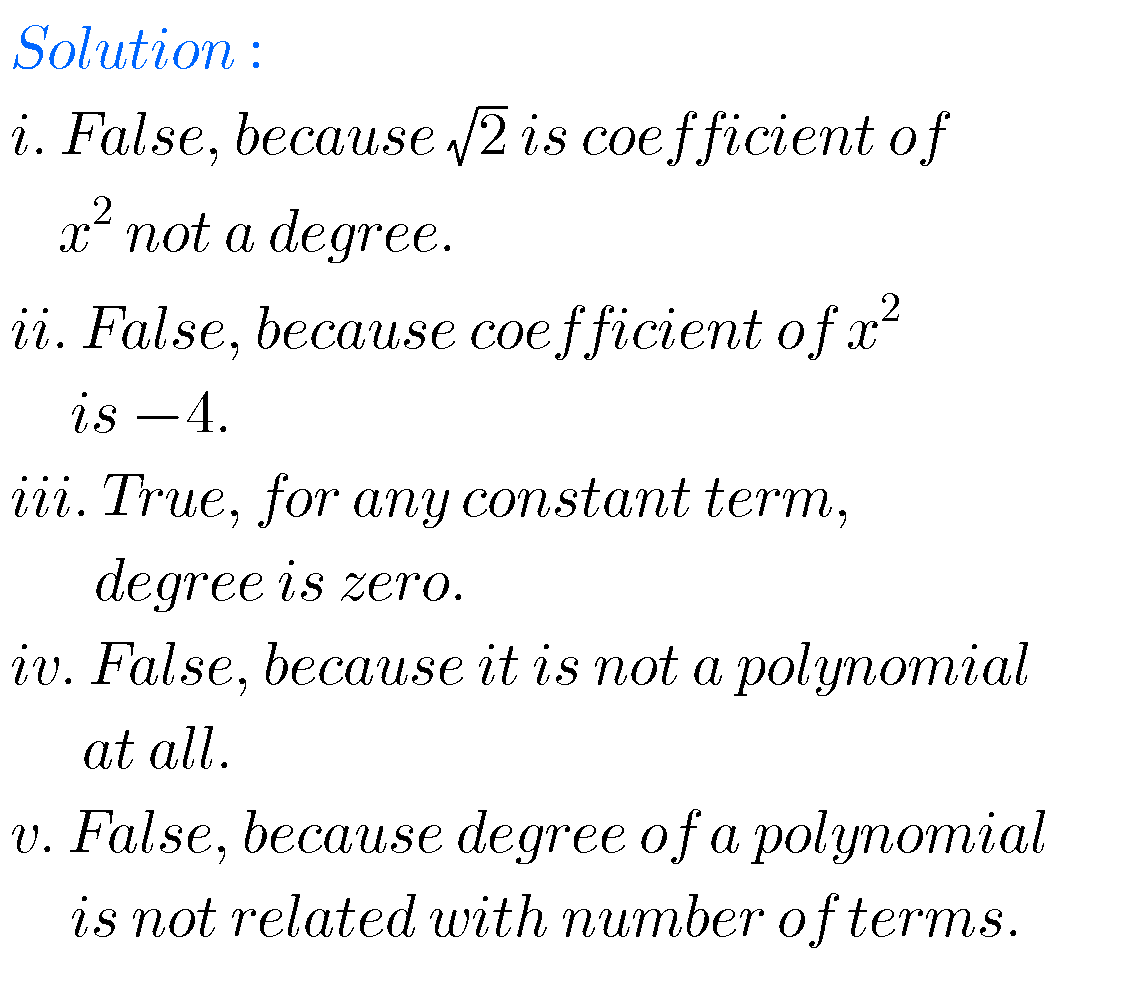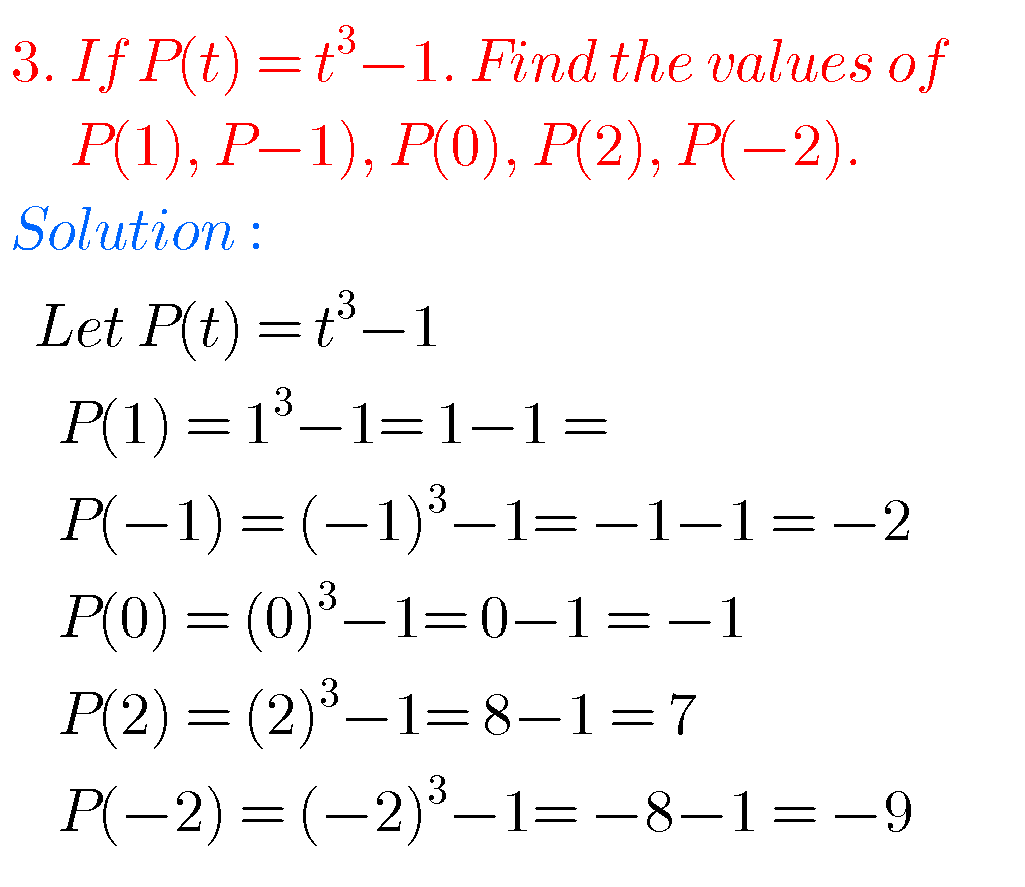SSC maths polynomials solutions

Exercise 3.2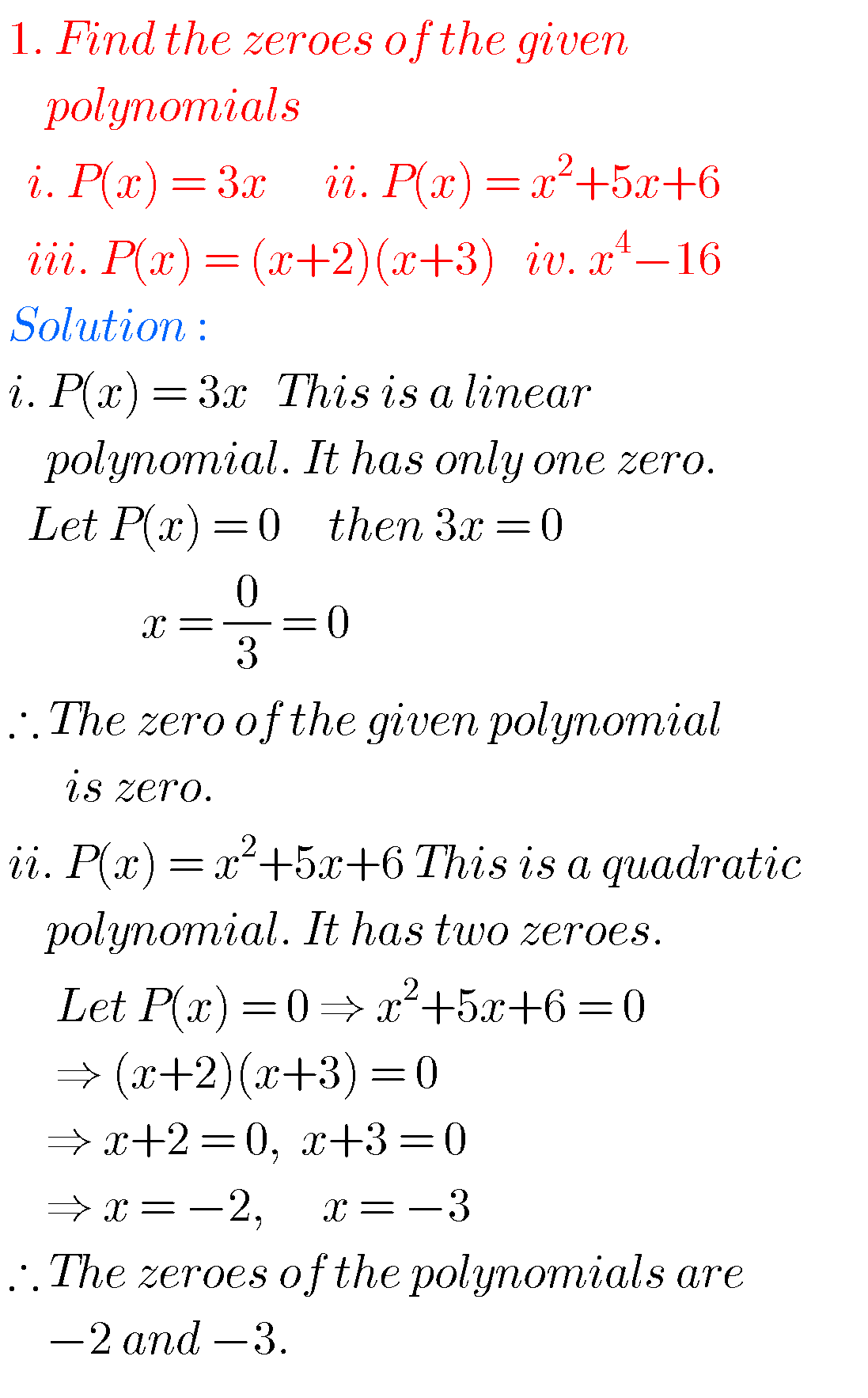iiii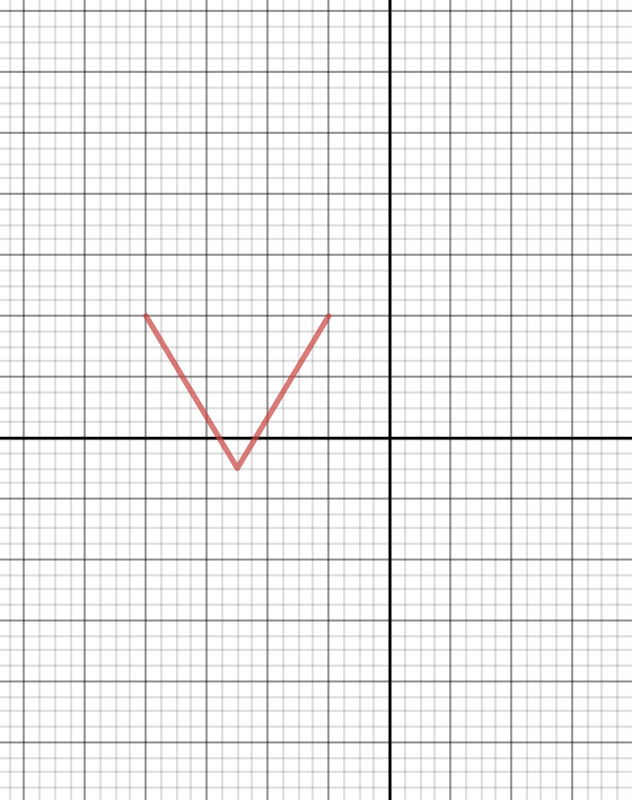iv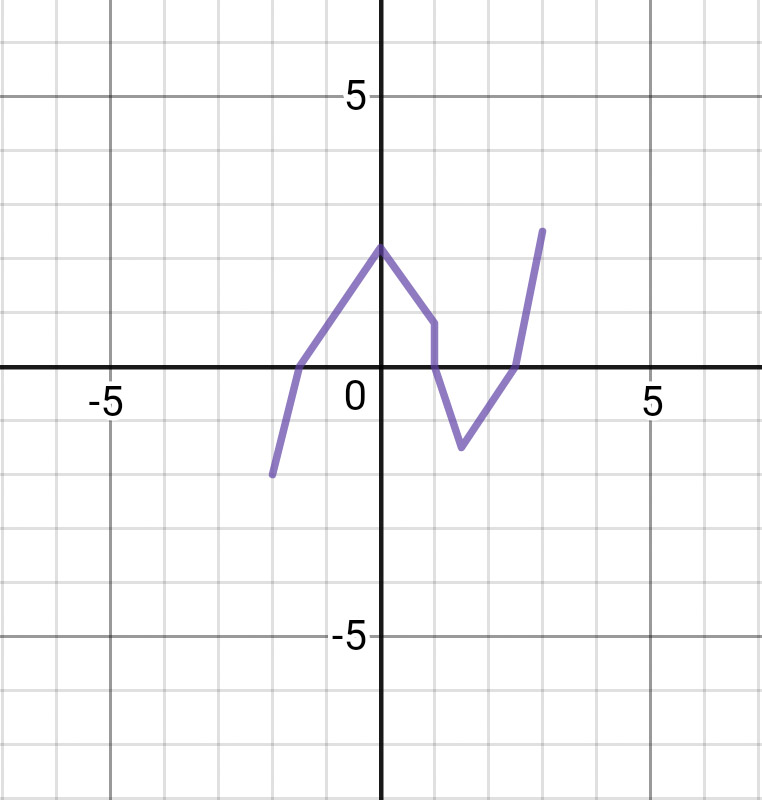Graphs : i, ii,   , iv, v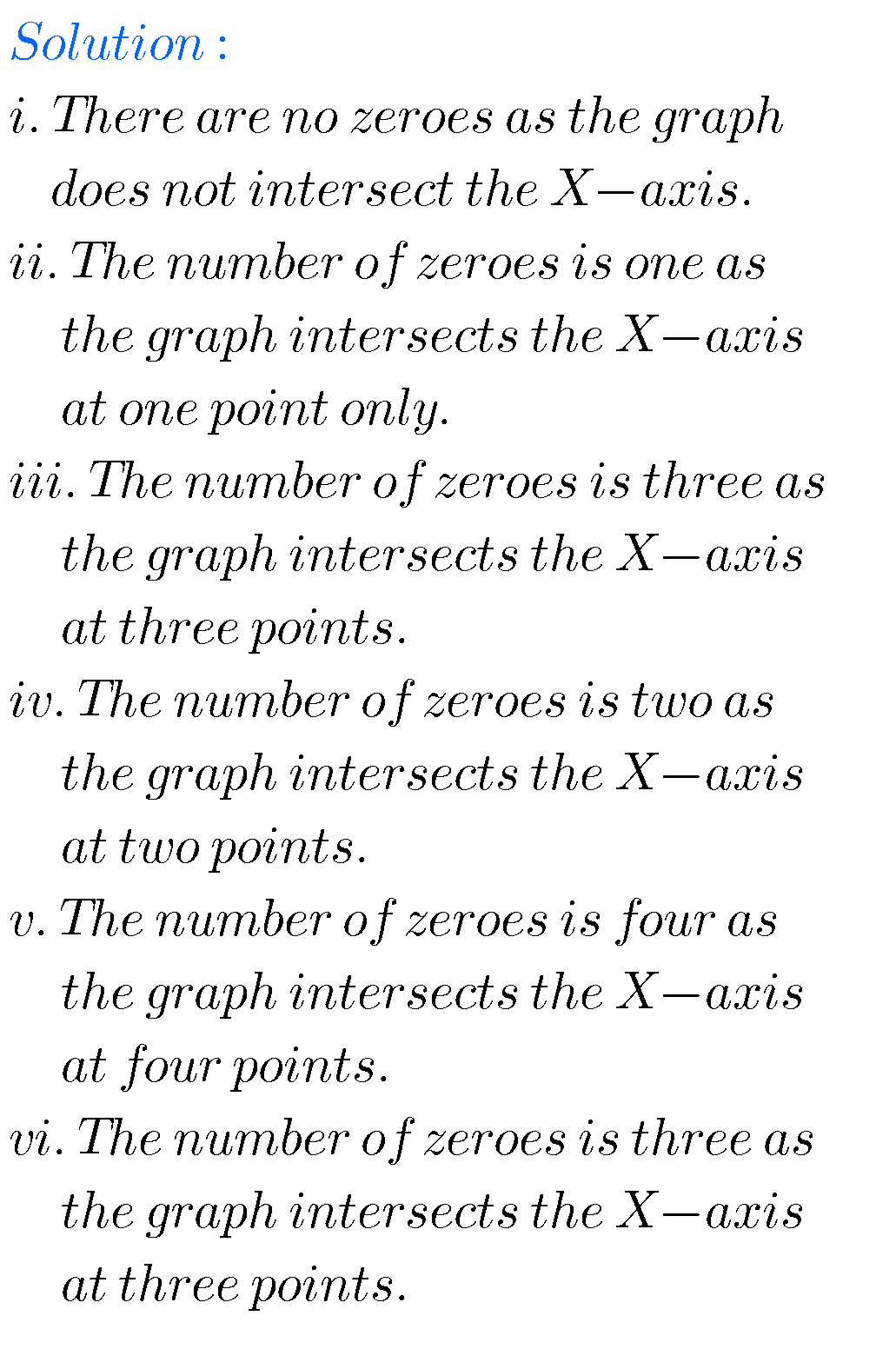Graph i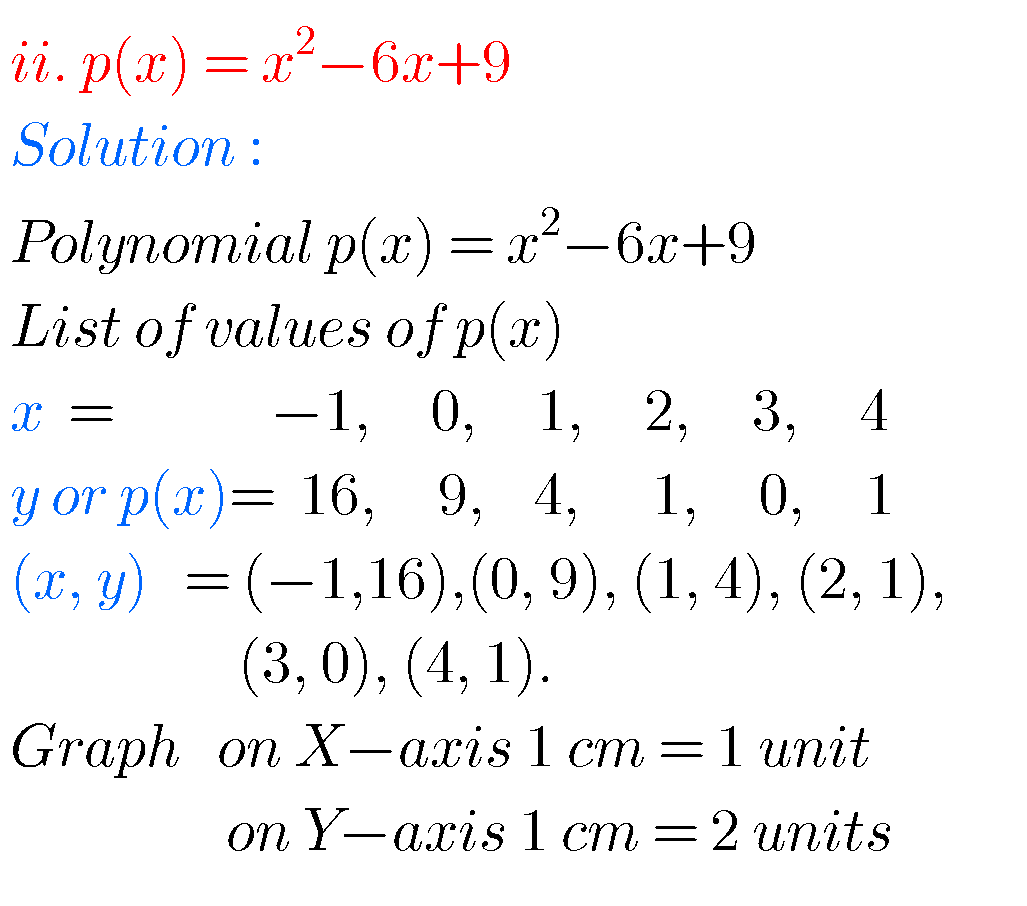Graph ii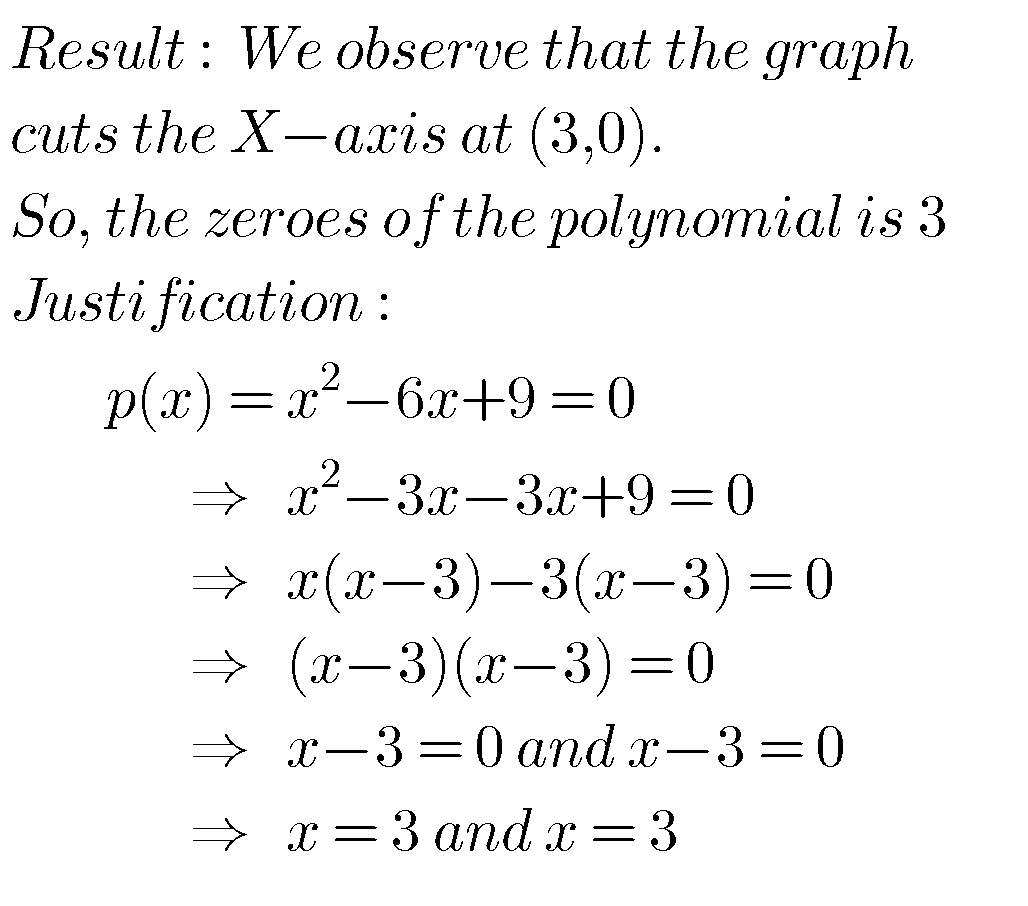Graph iii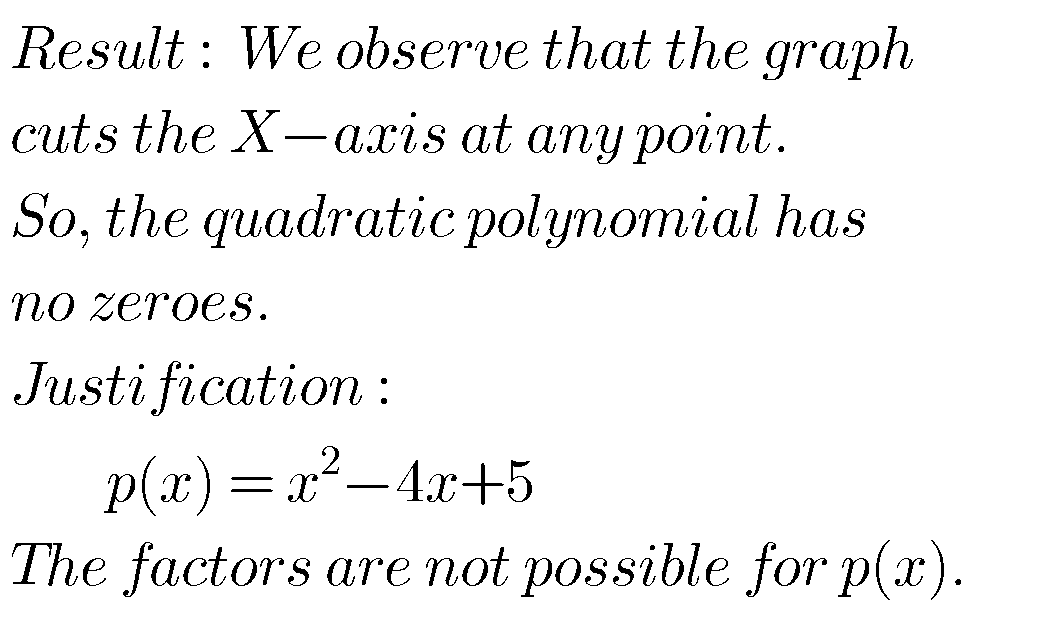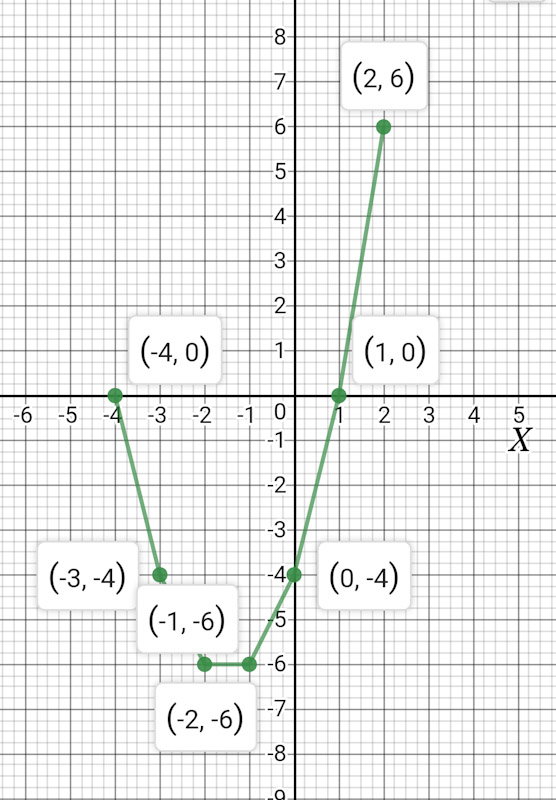Graph iv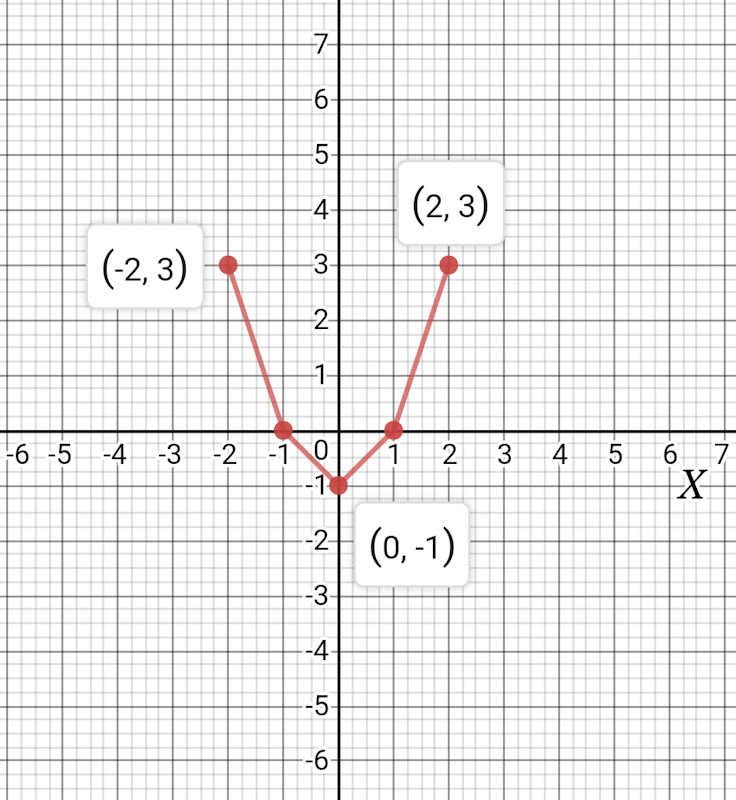Graph v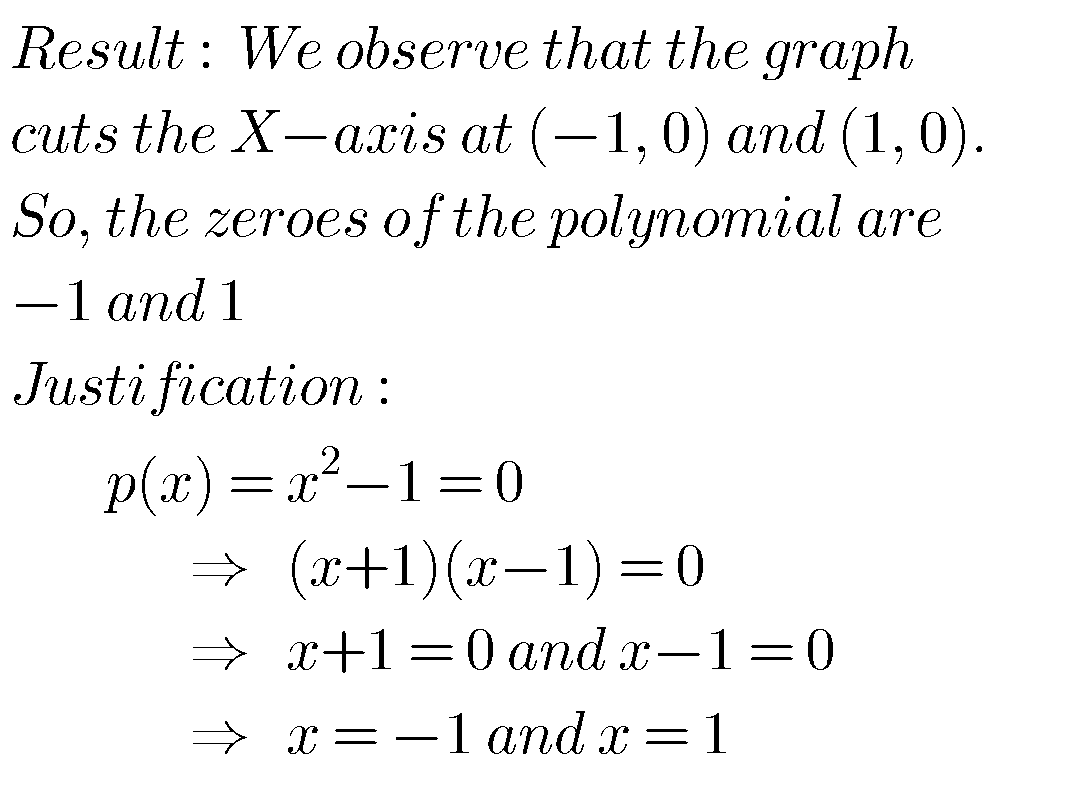Maths class X polynomials solutions

Exercise 3.3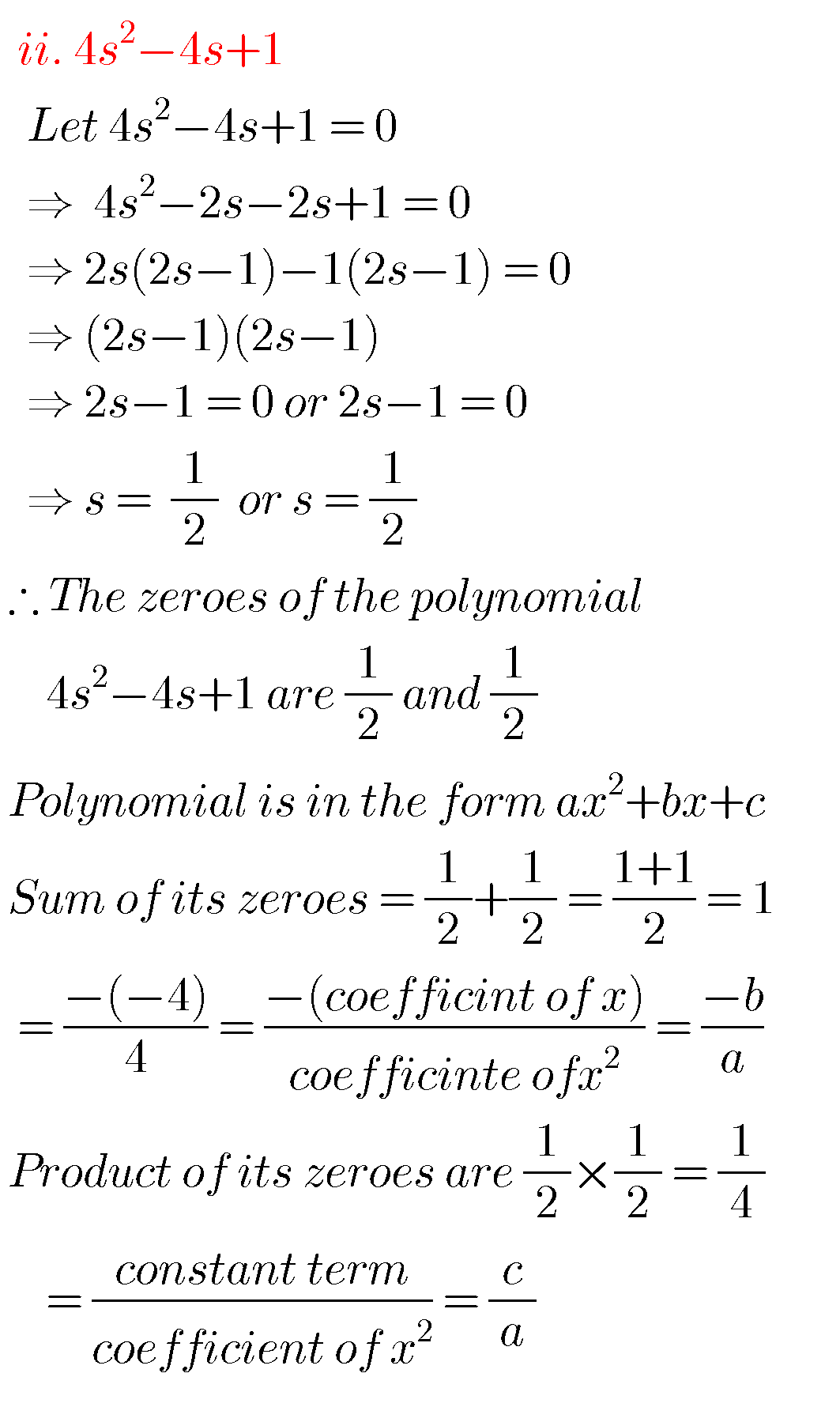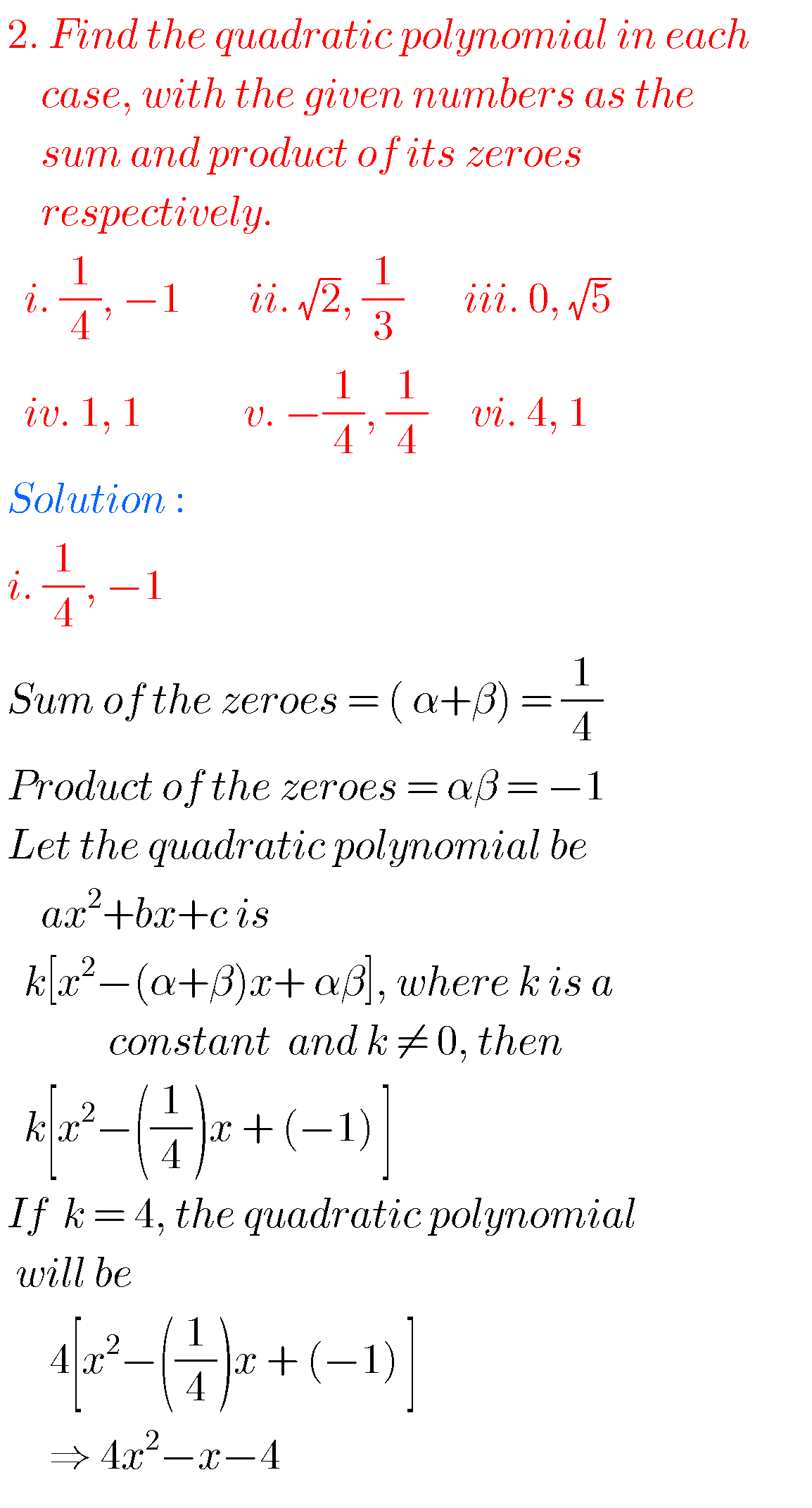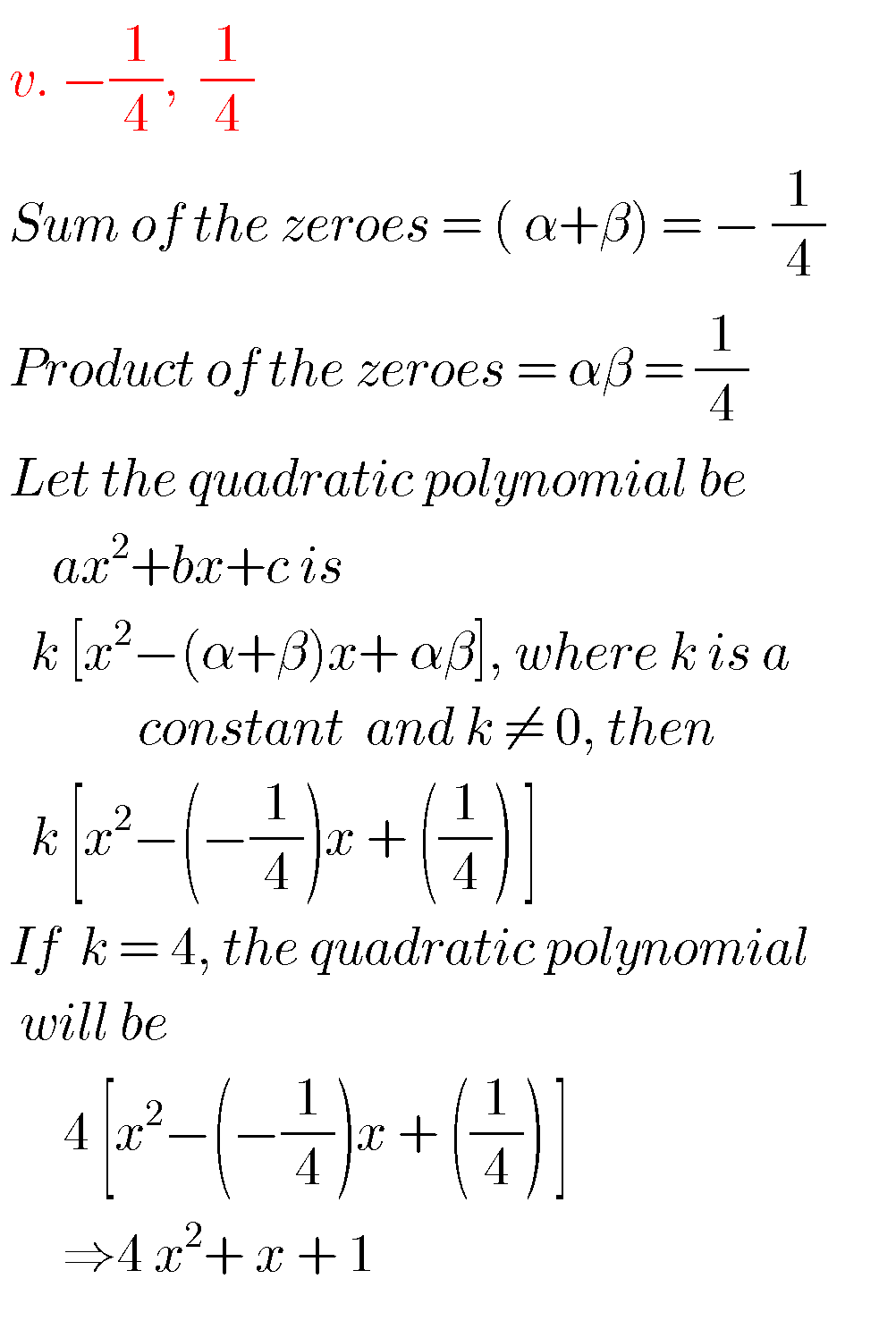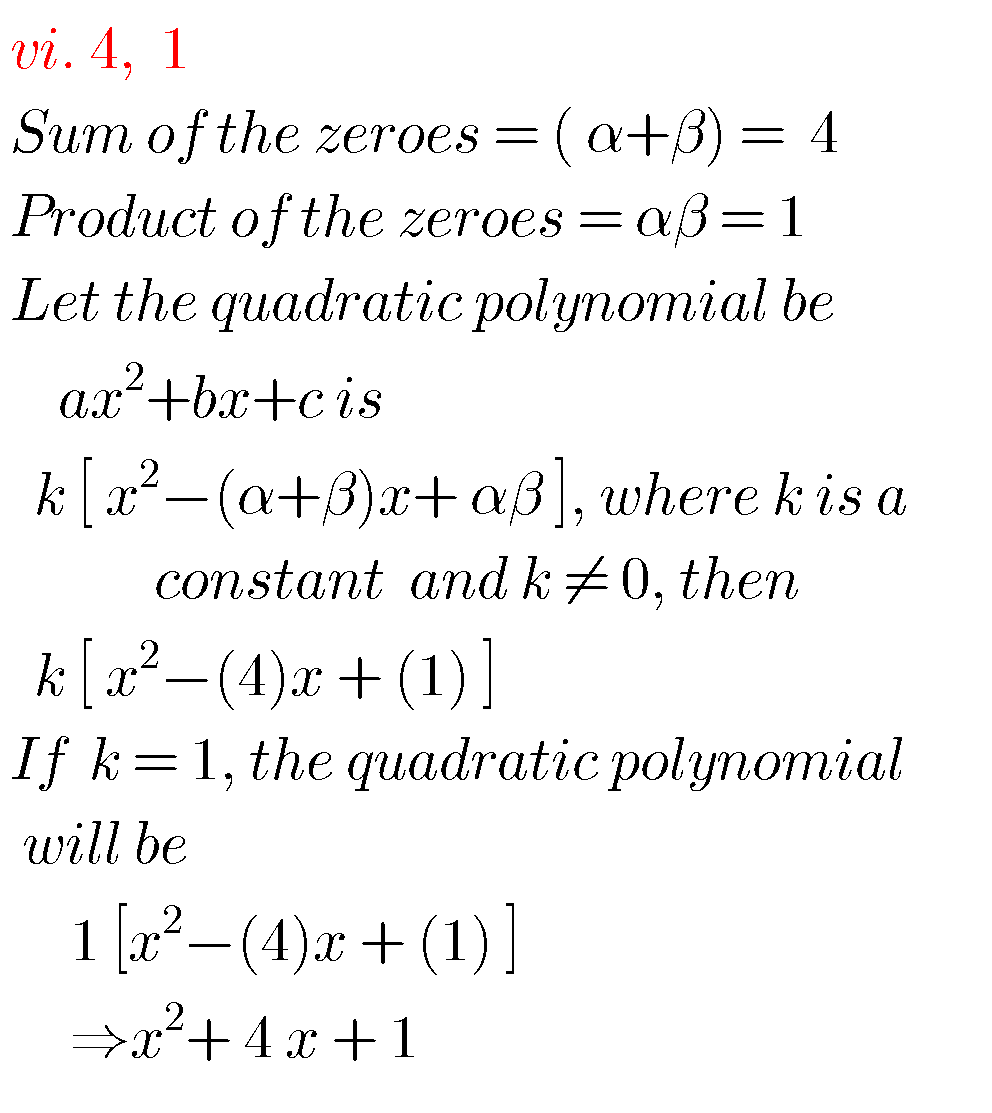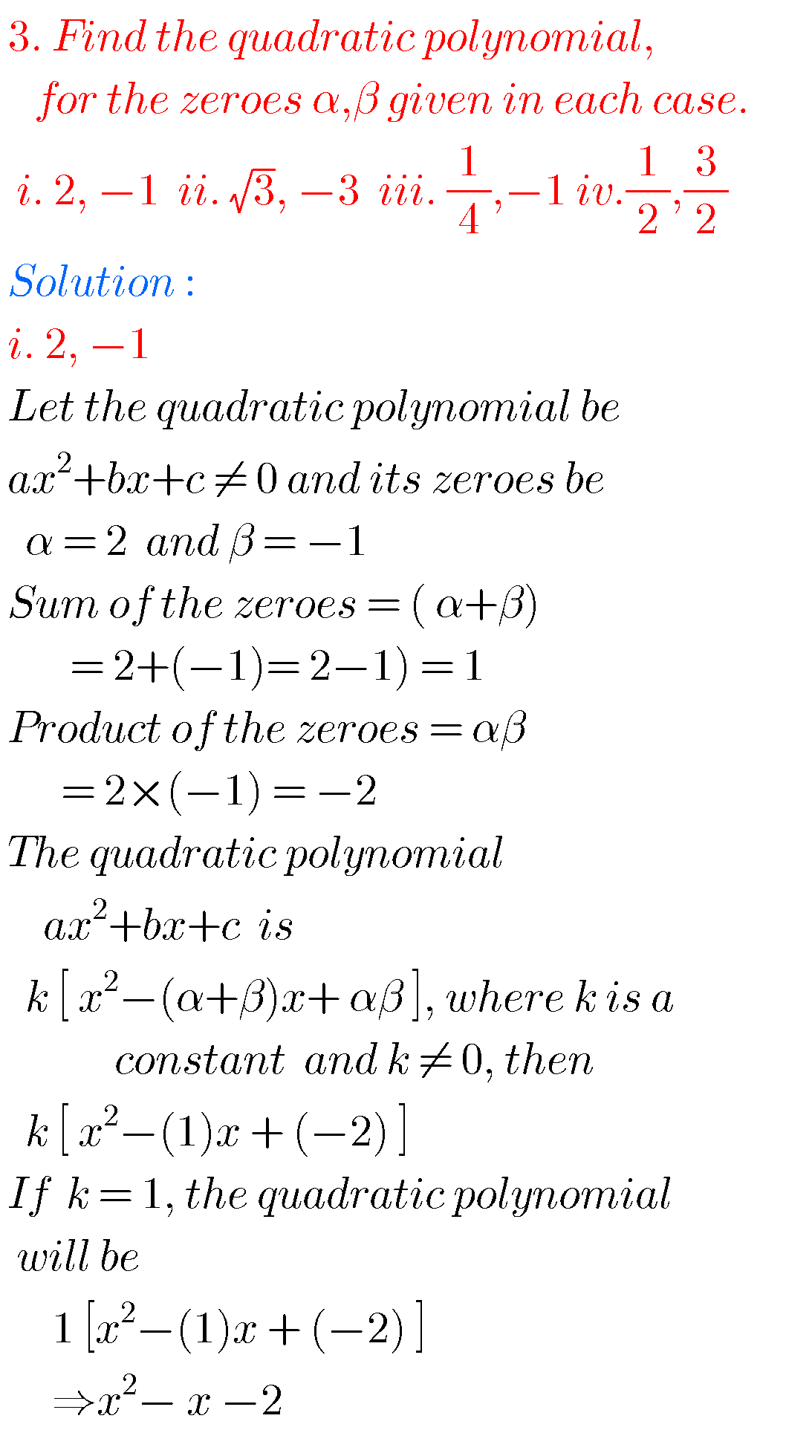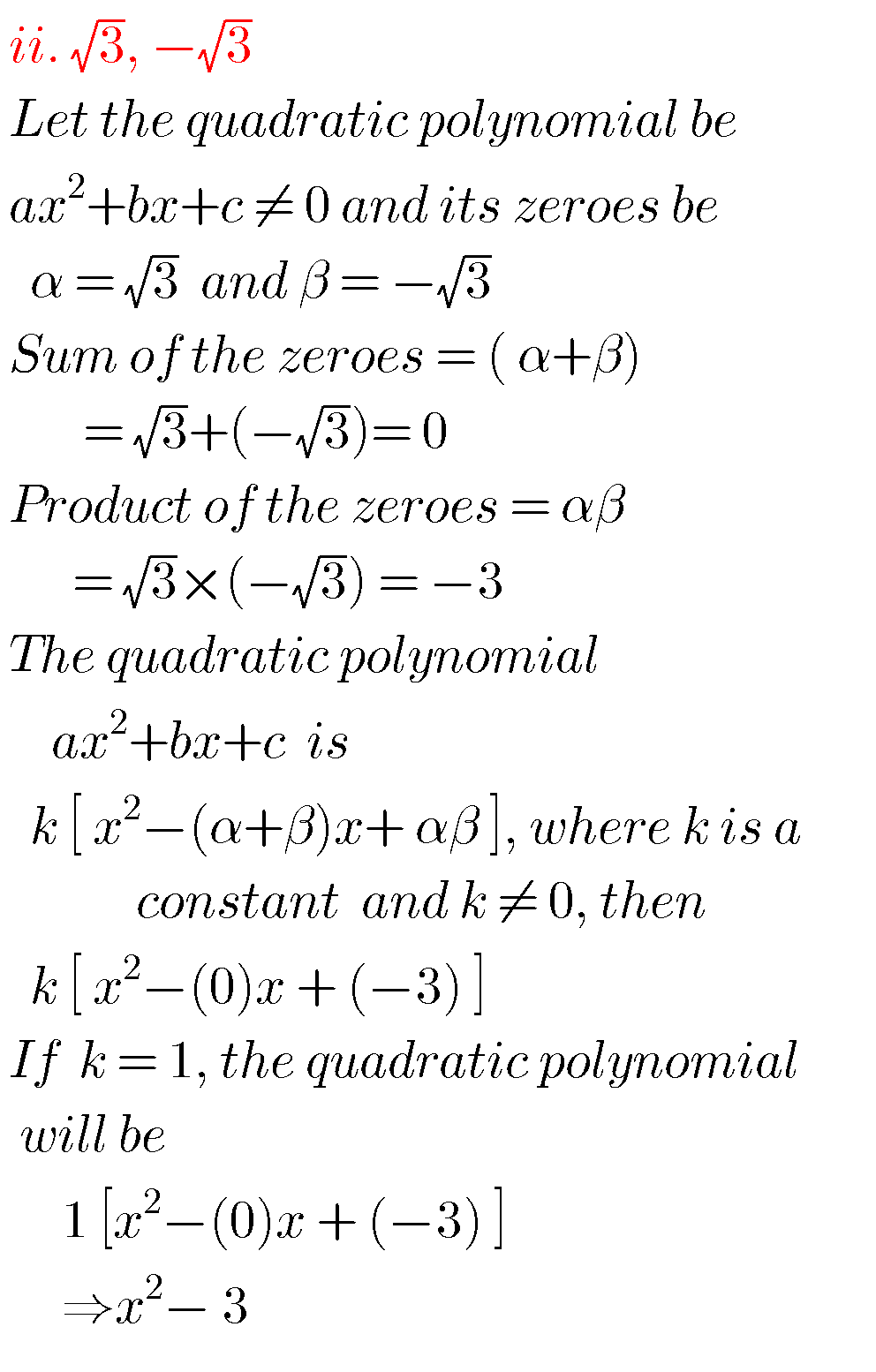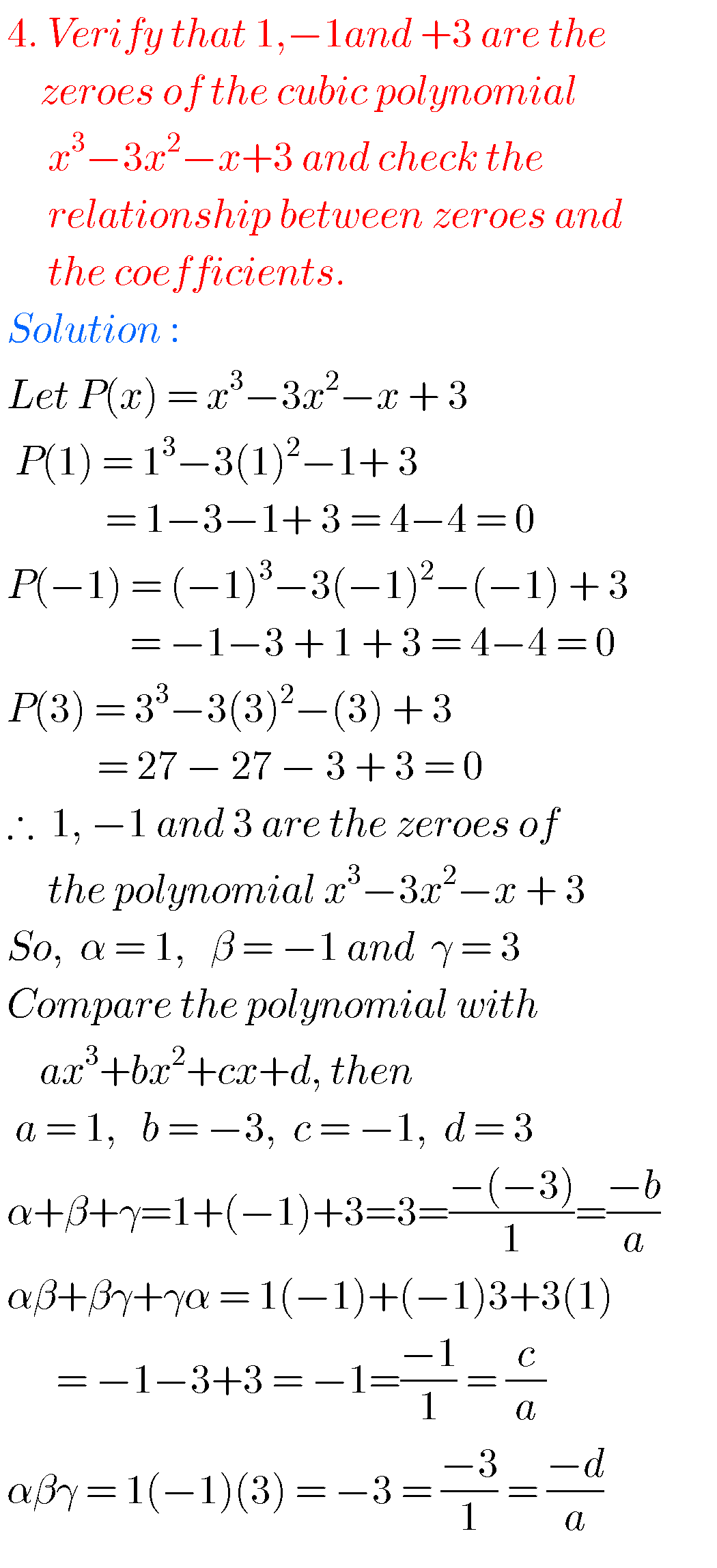10th class maths polynomials solutions

Exercise 3.4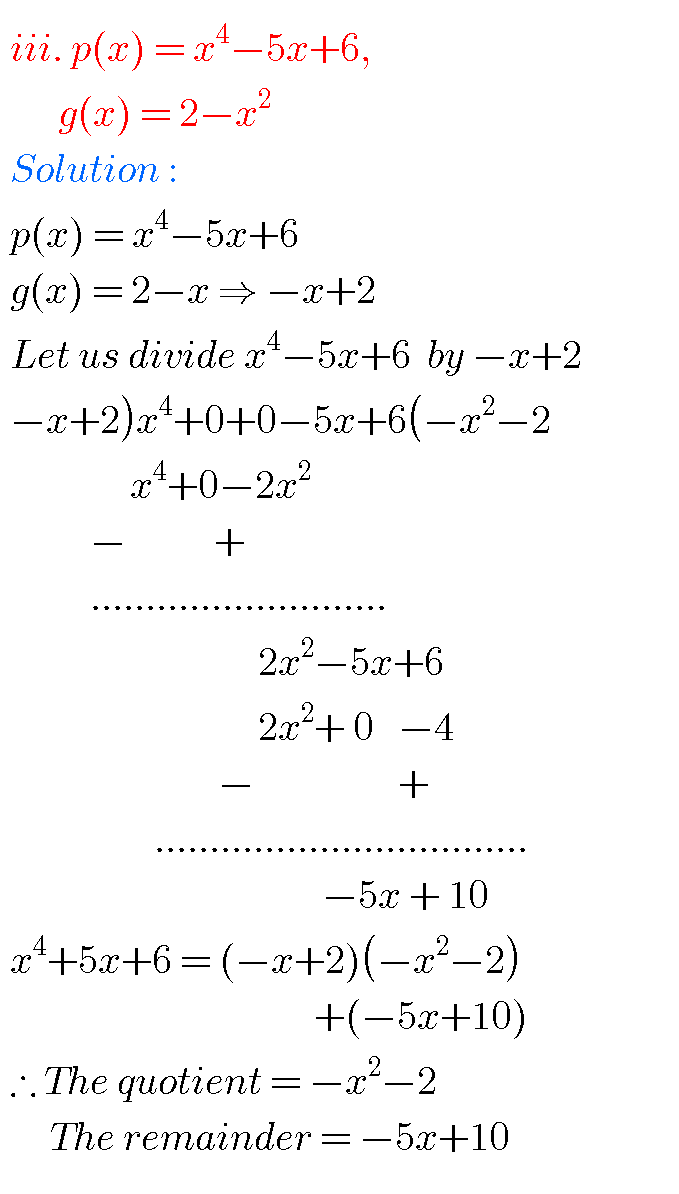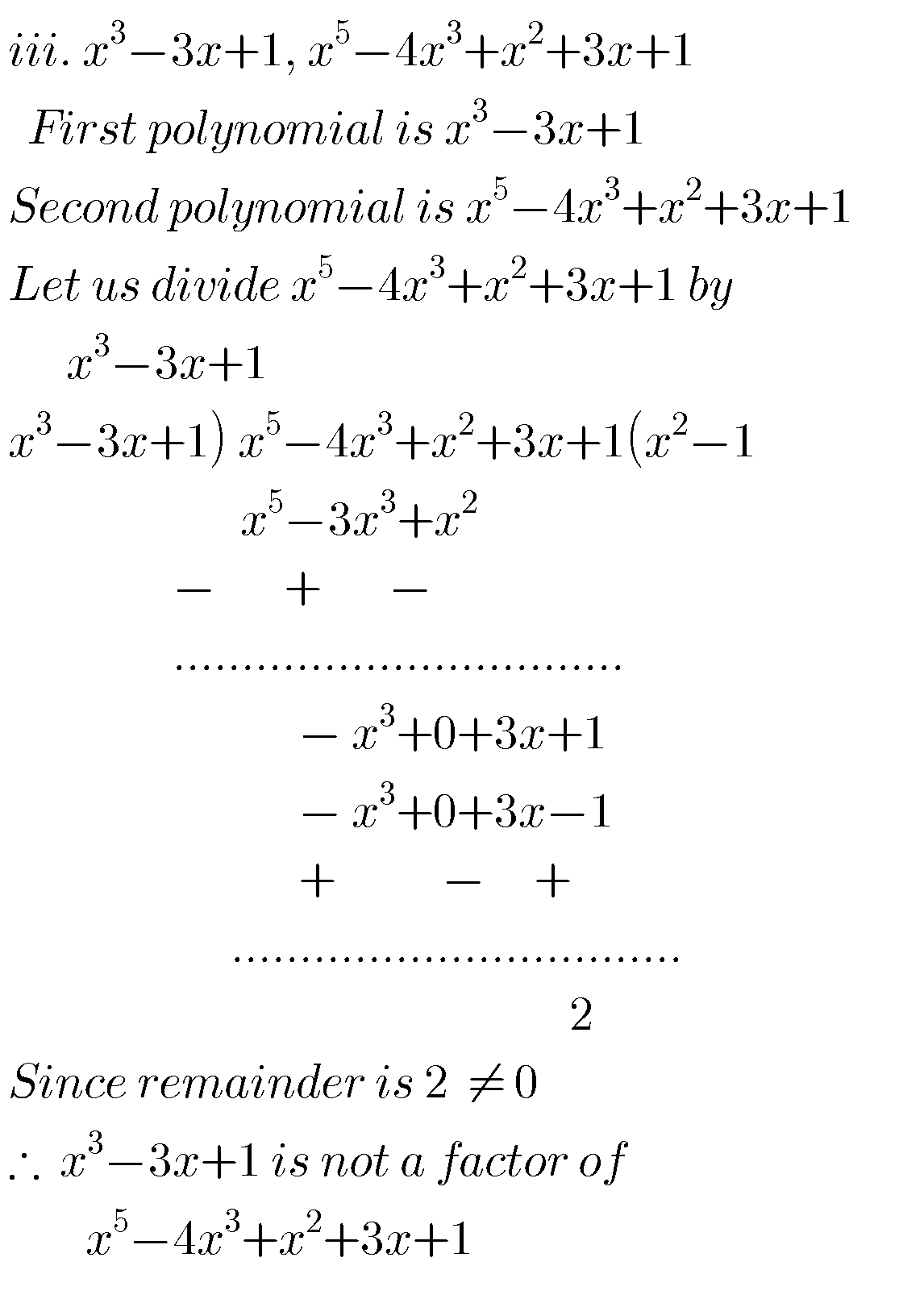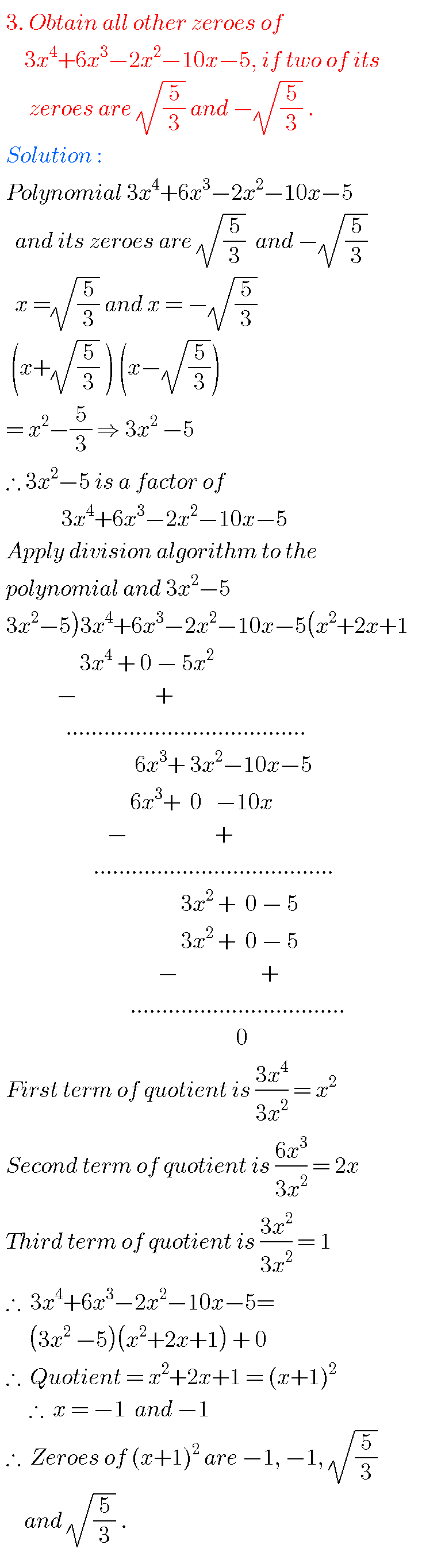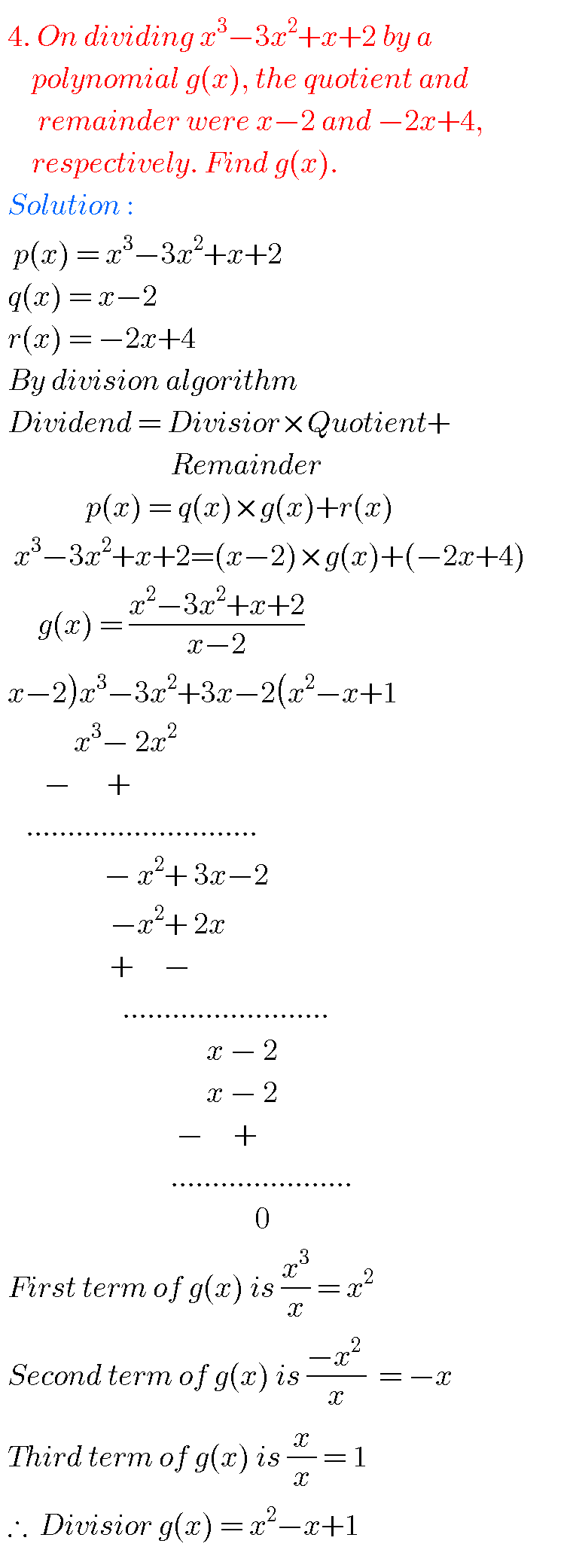Polynomials class 10 maths

Optinal exercise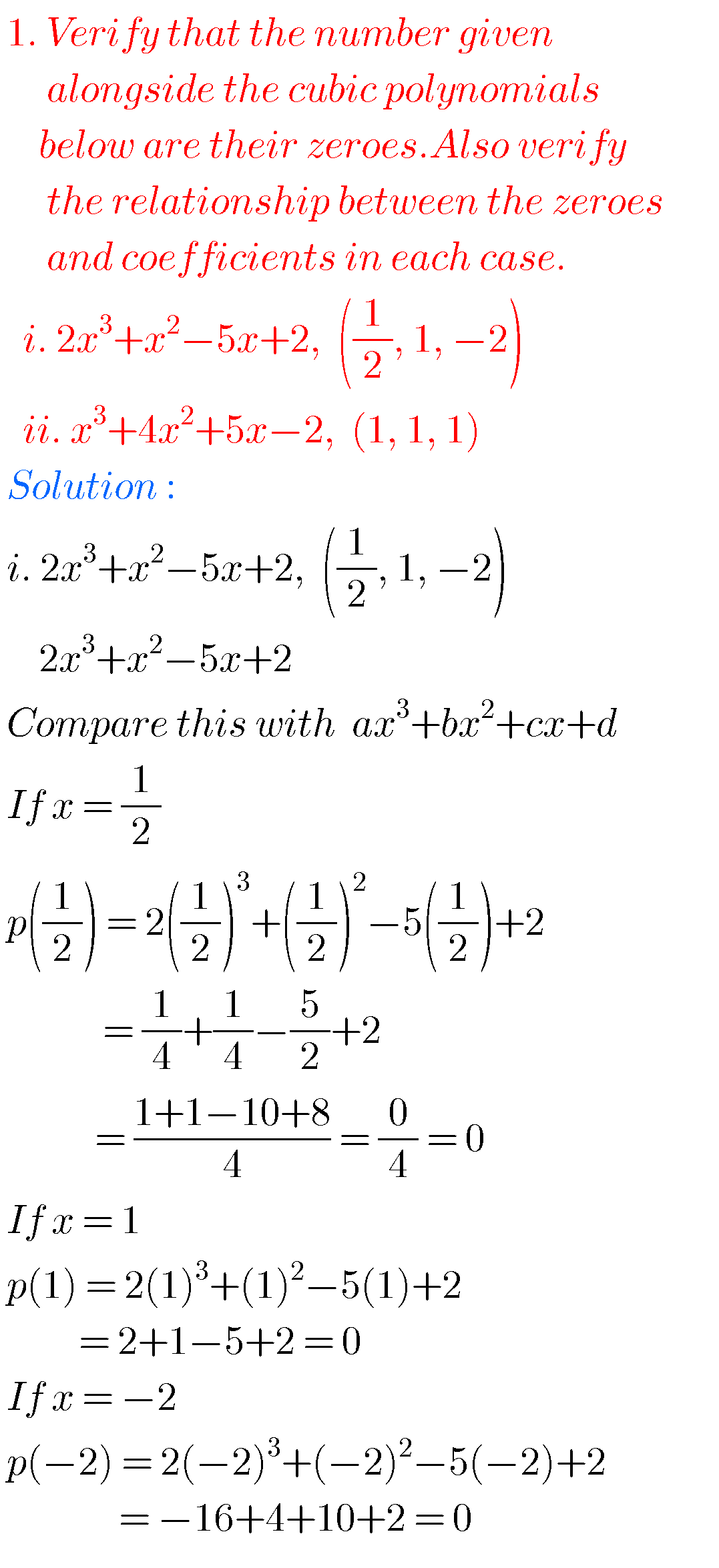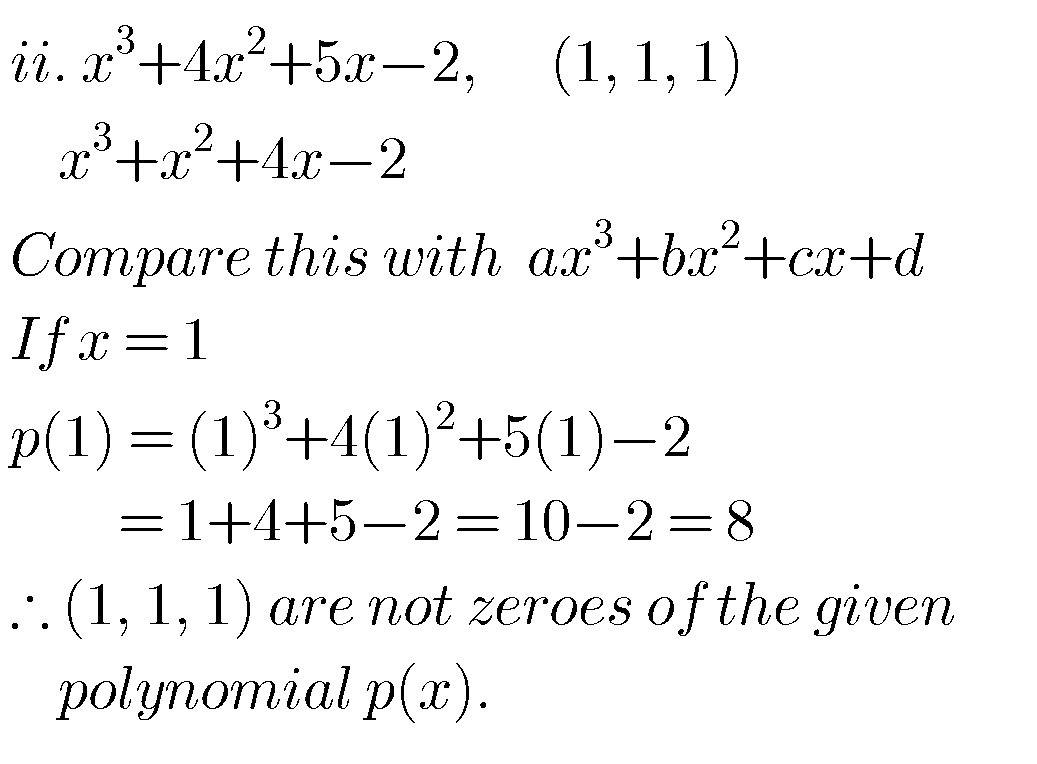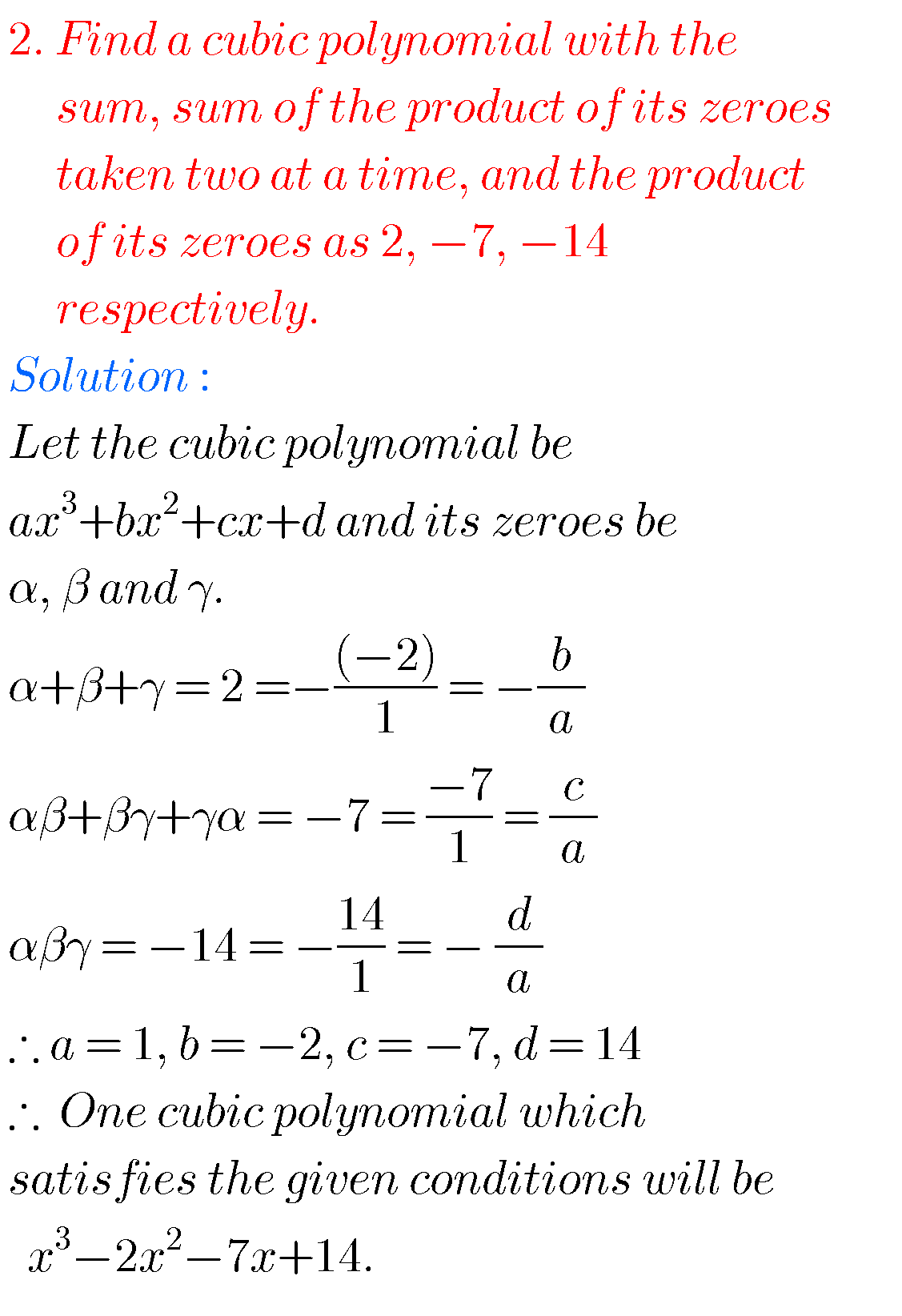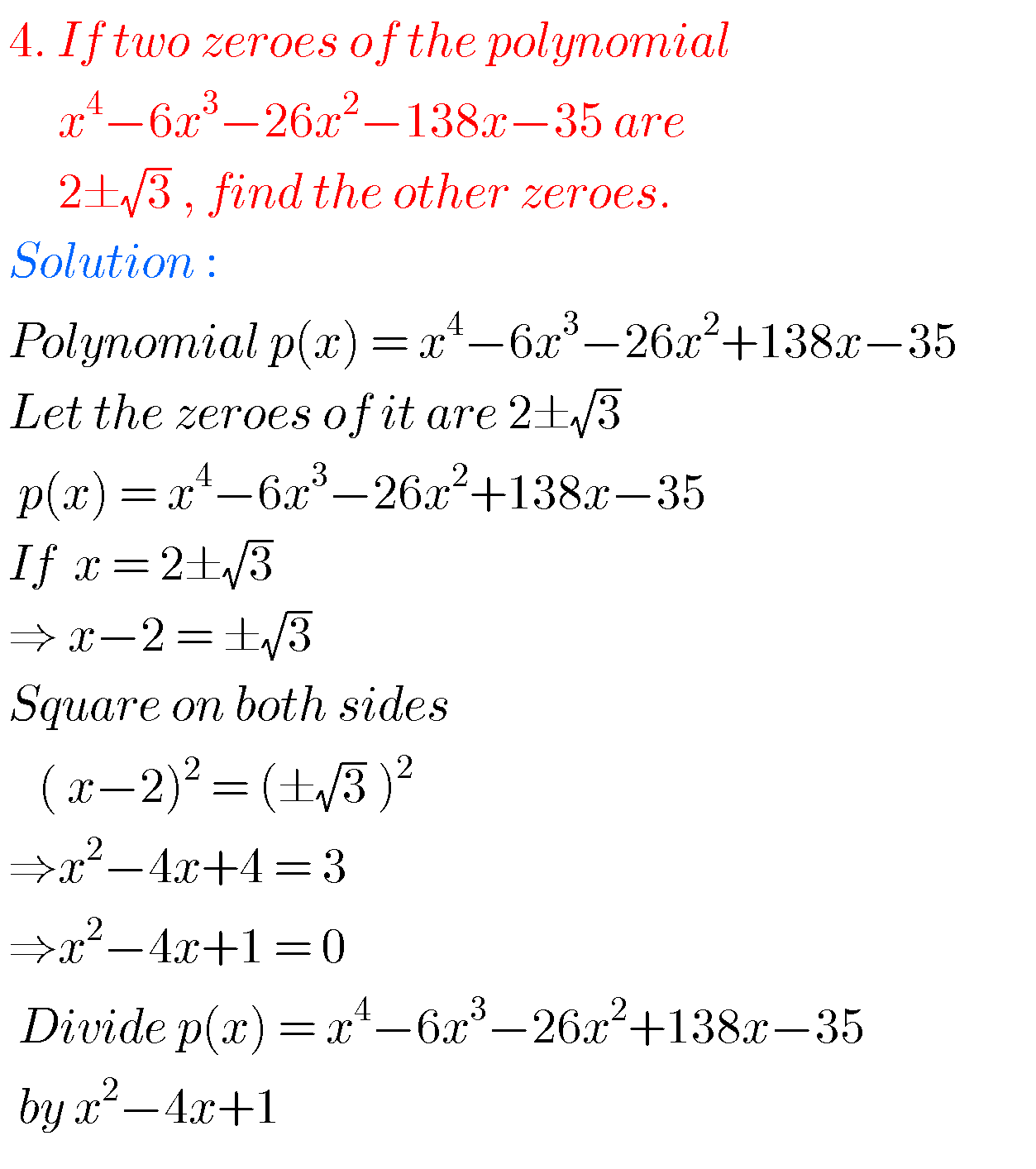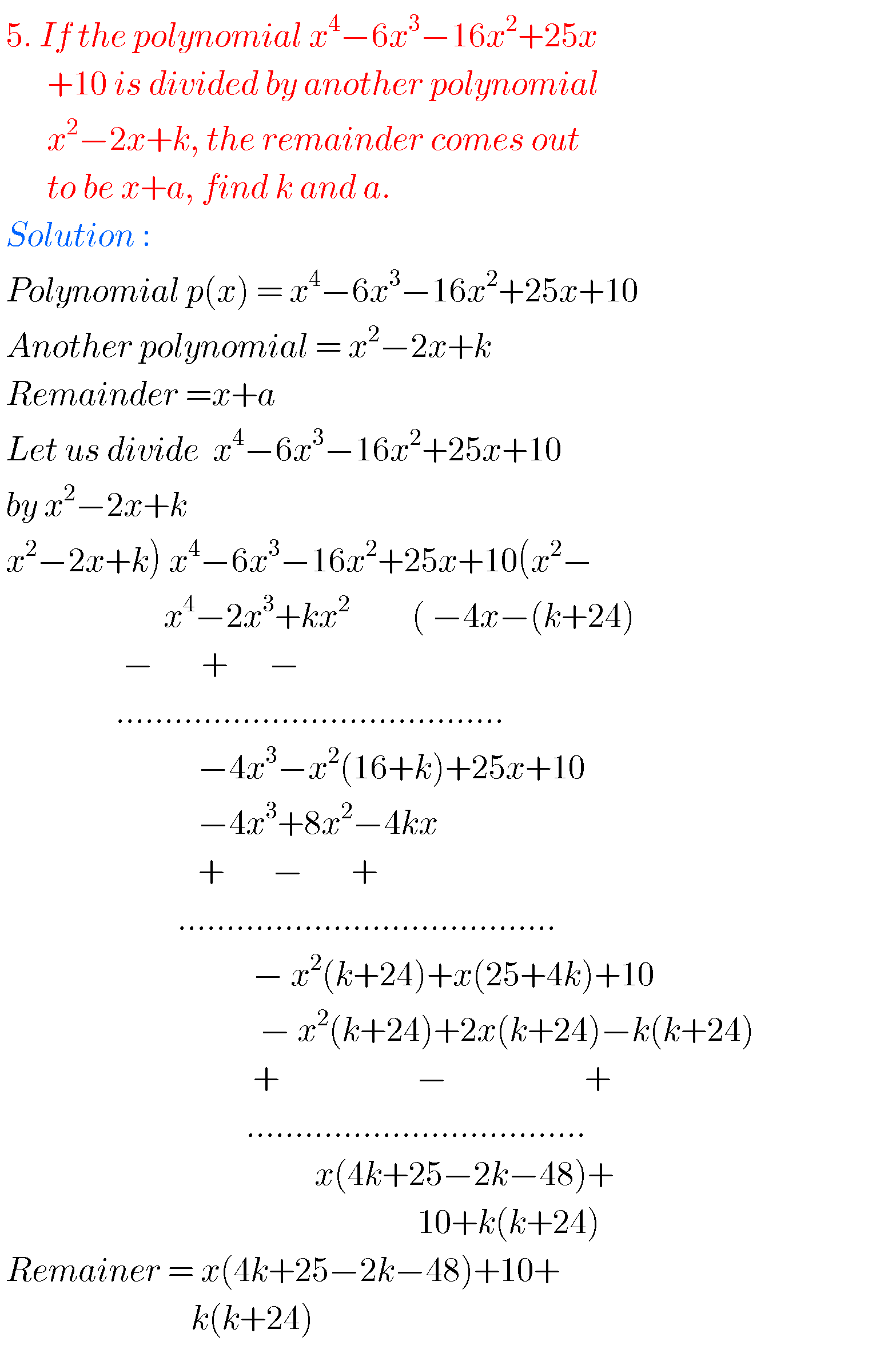Some more solutions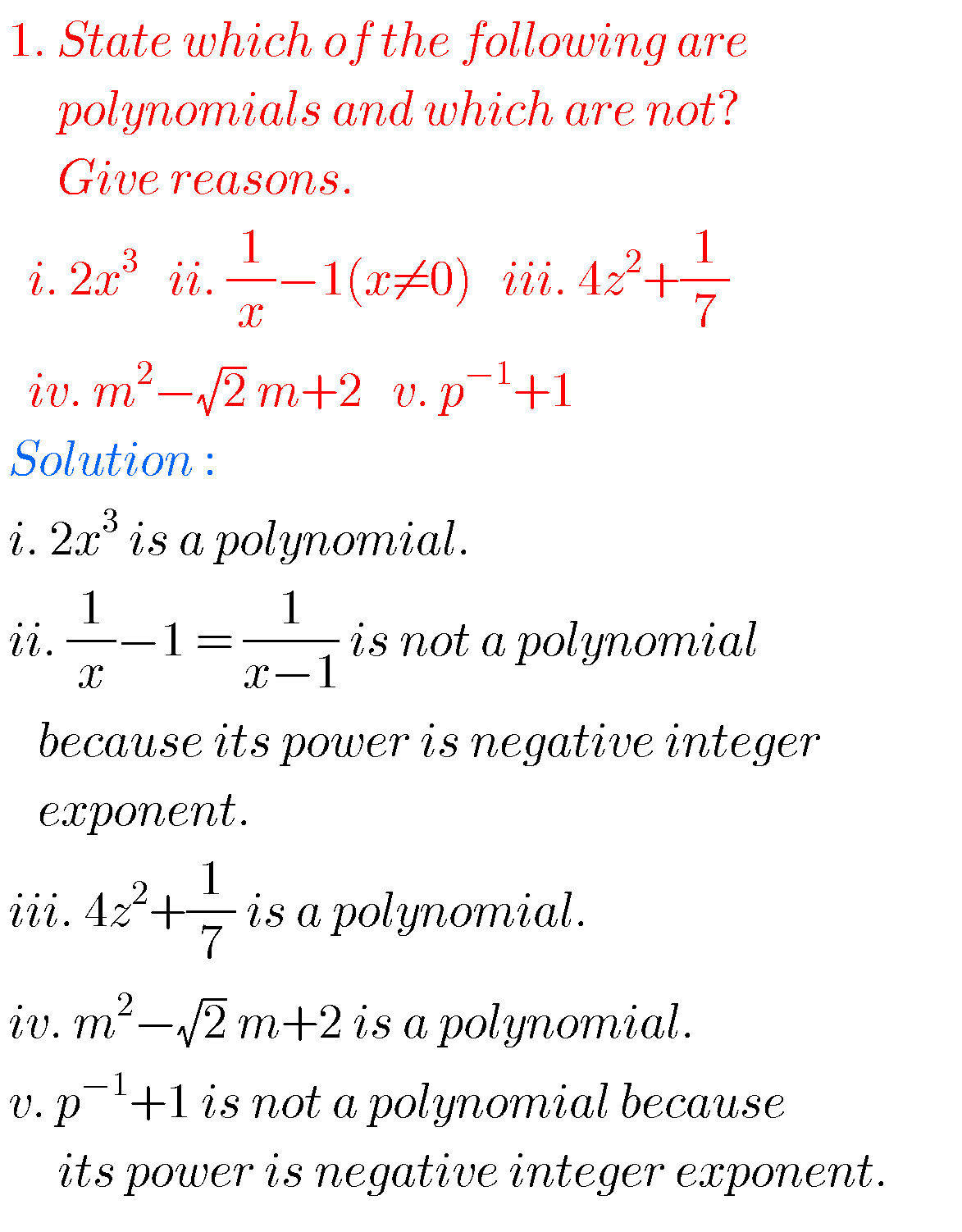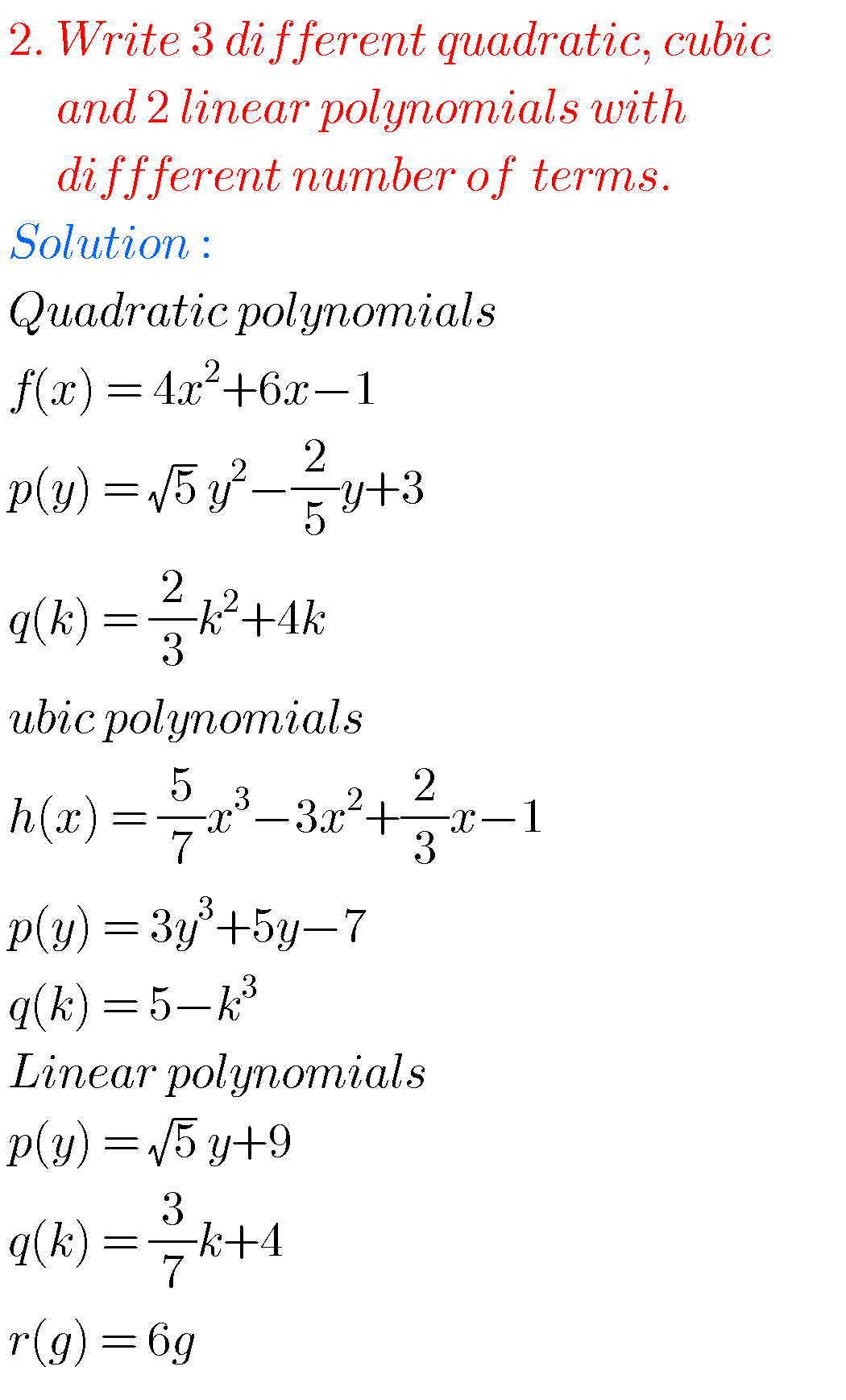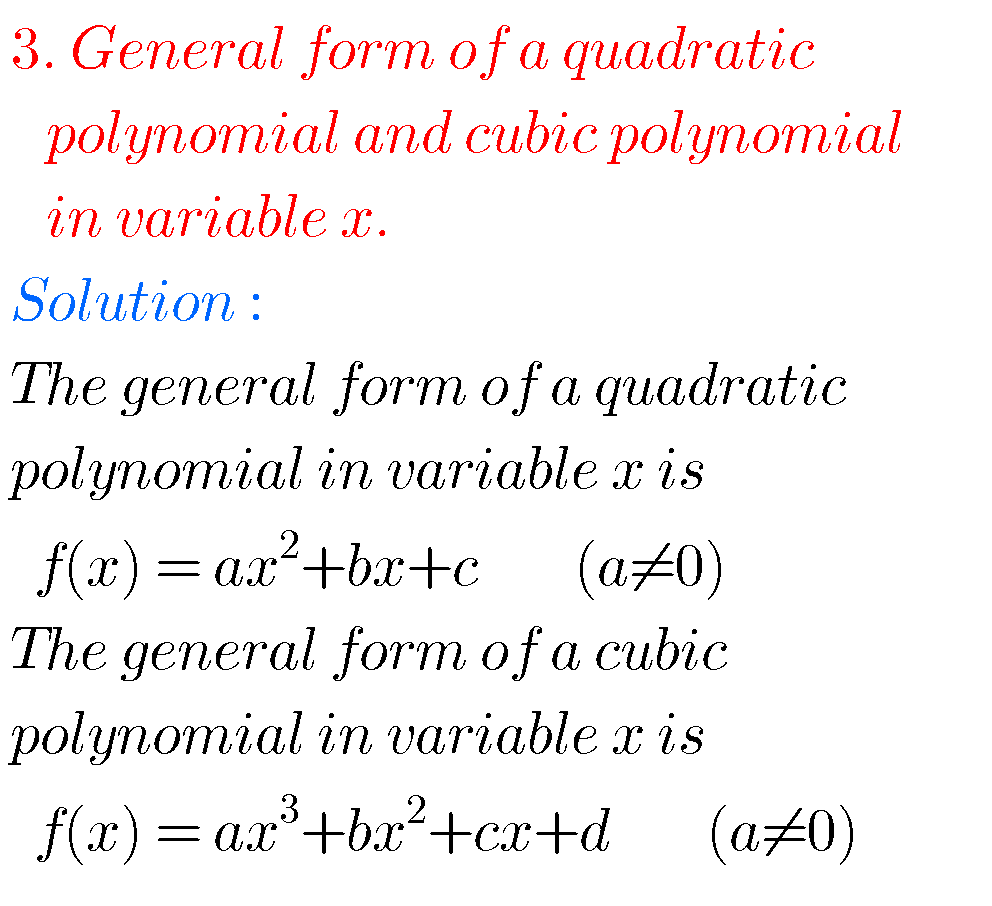4.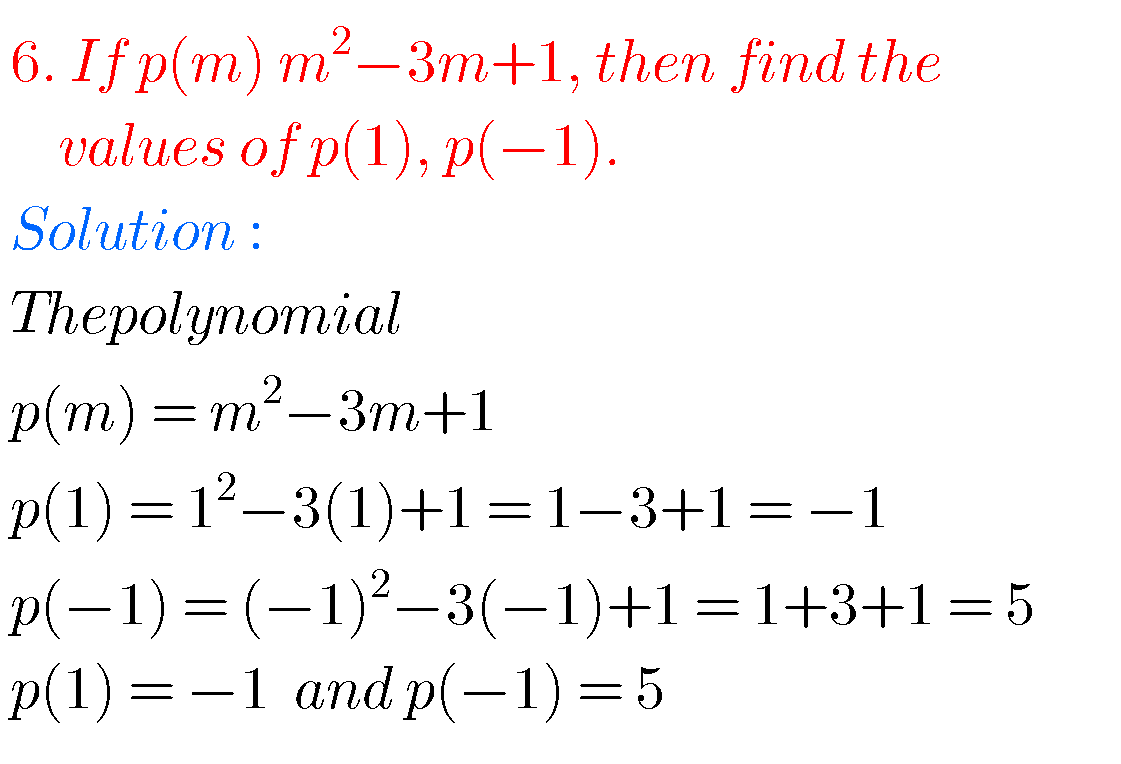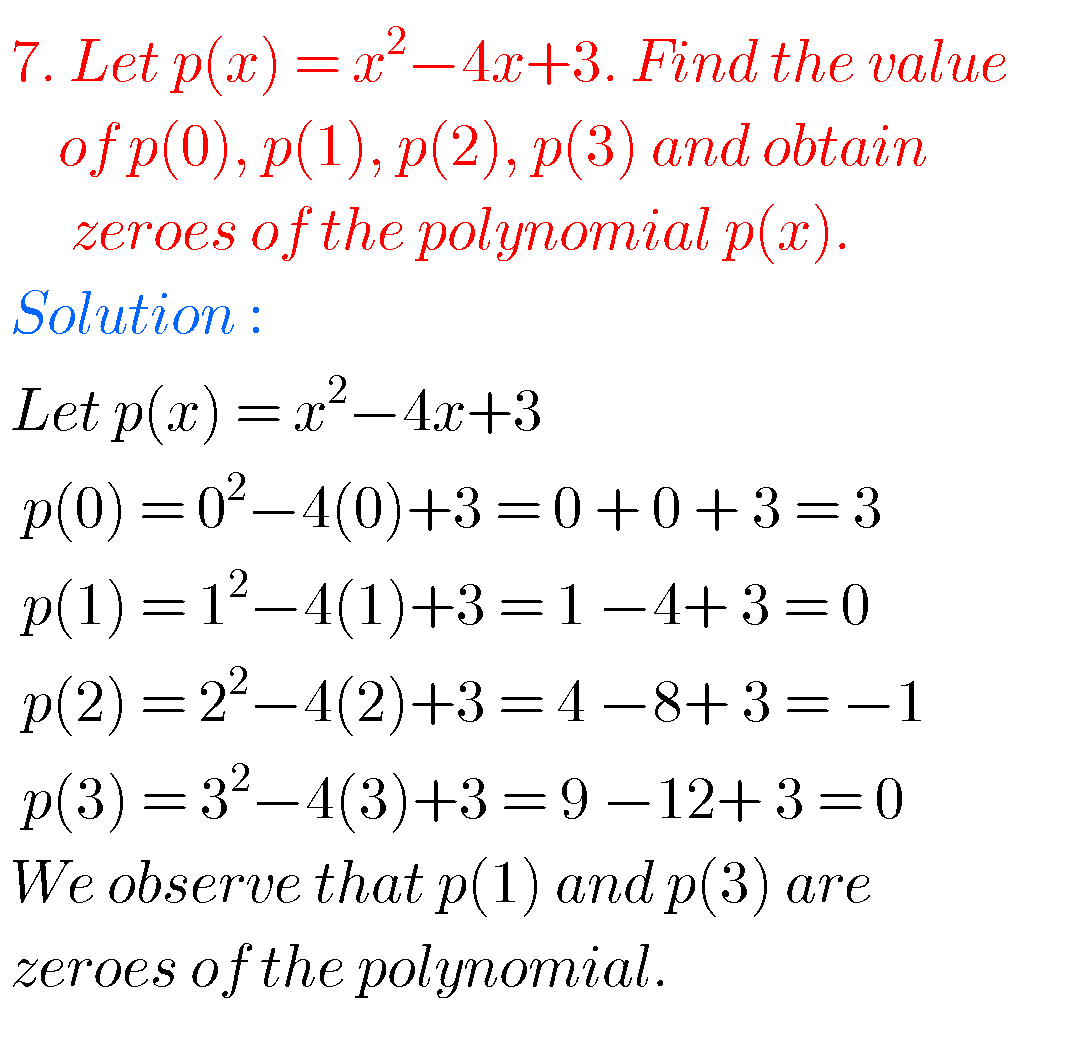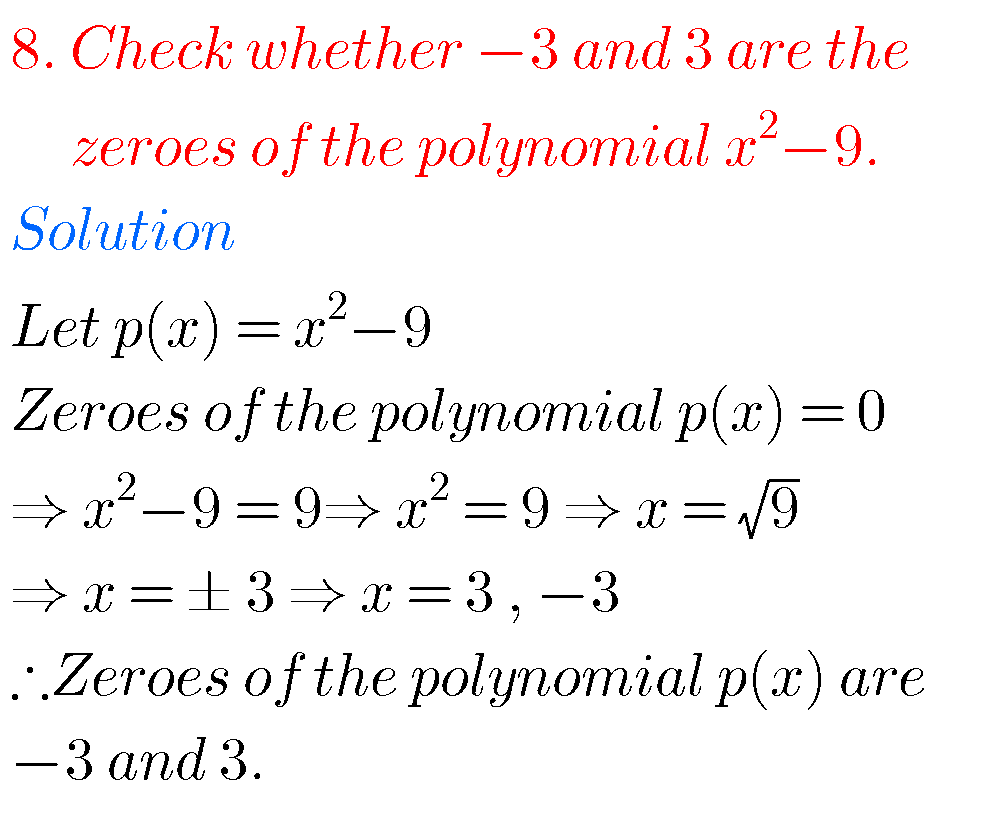Graph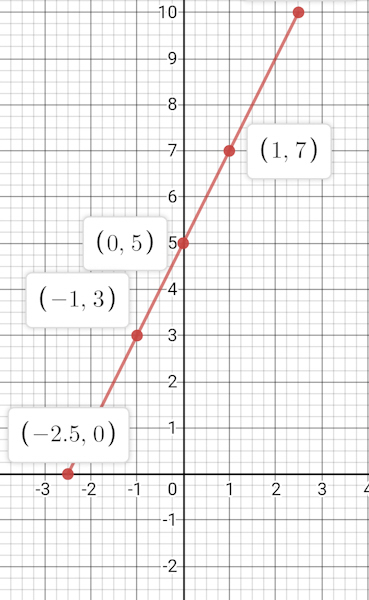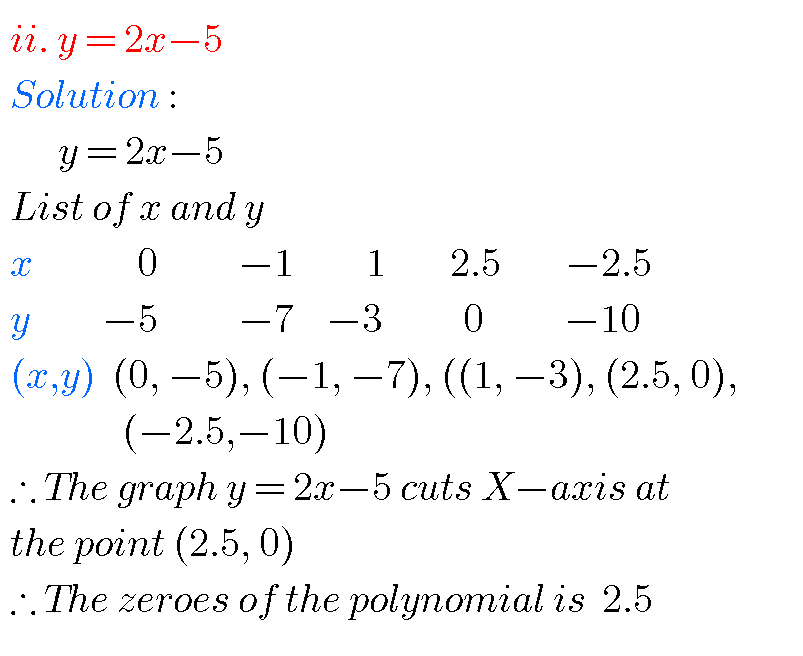Graph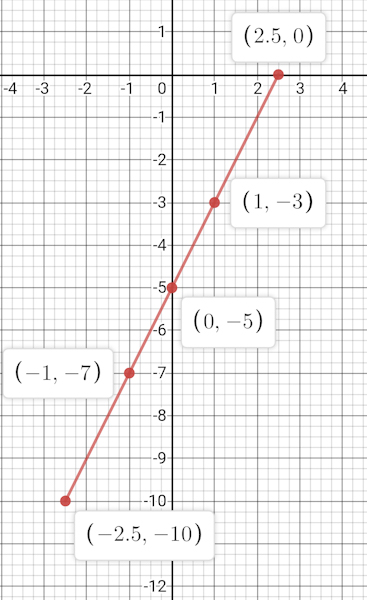Graph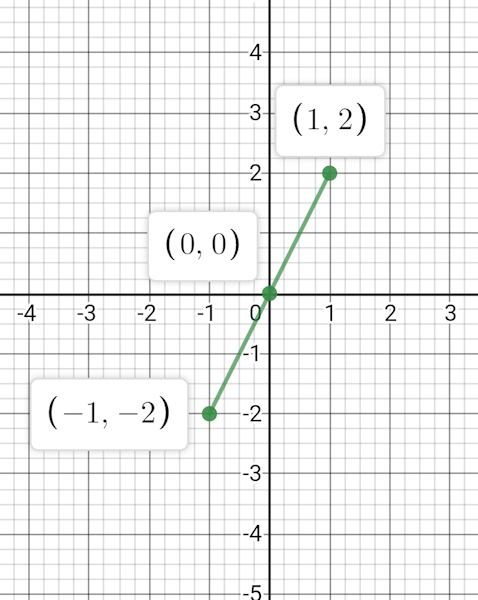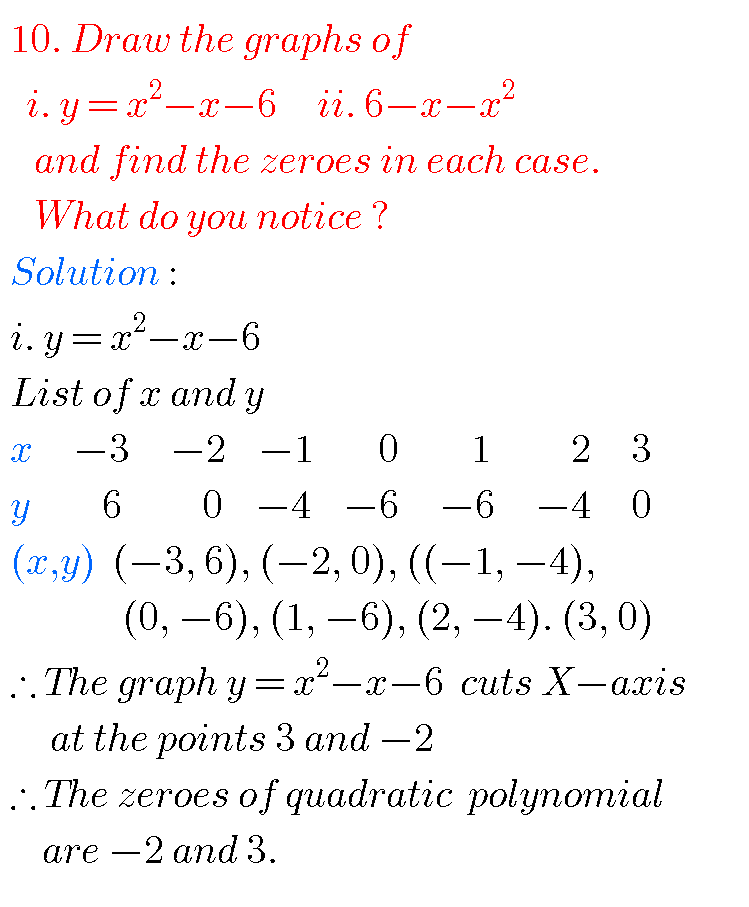Graph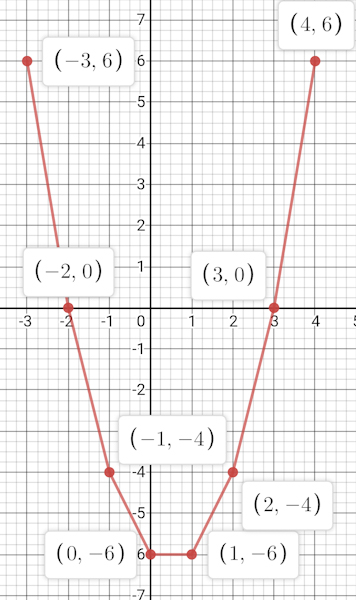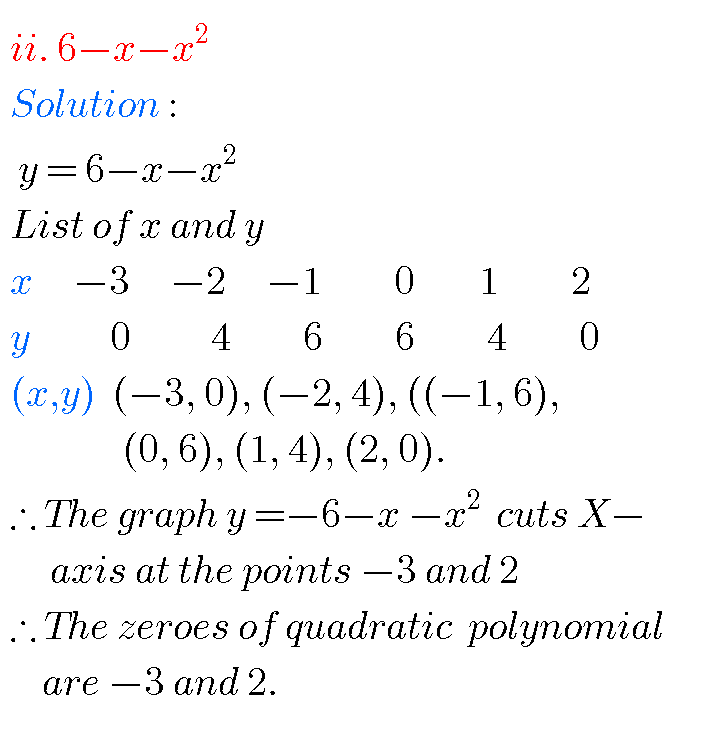Graph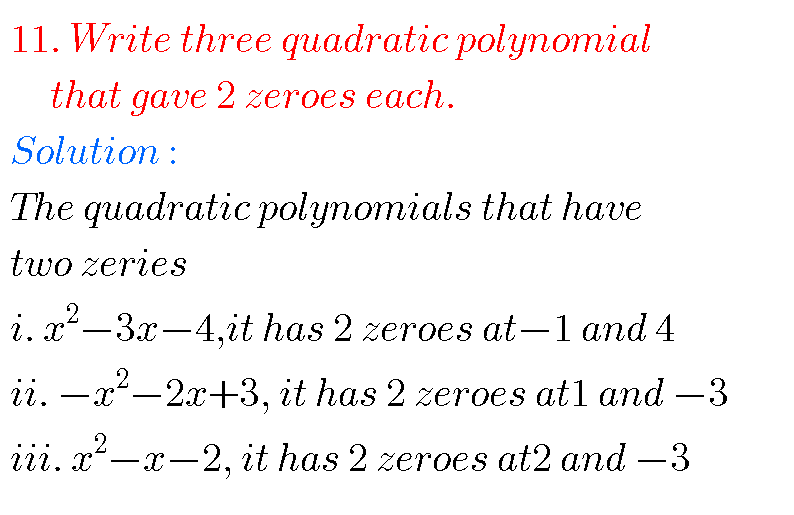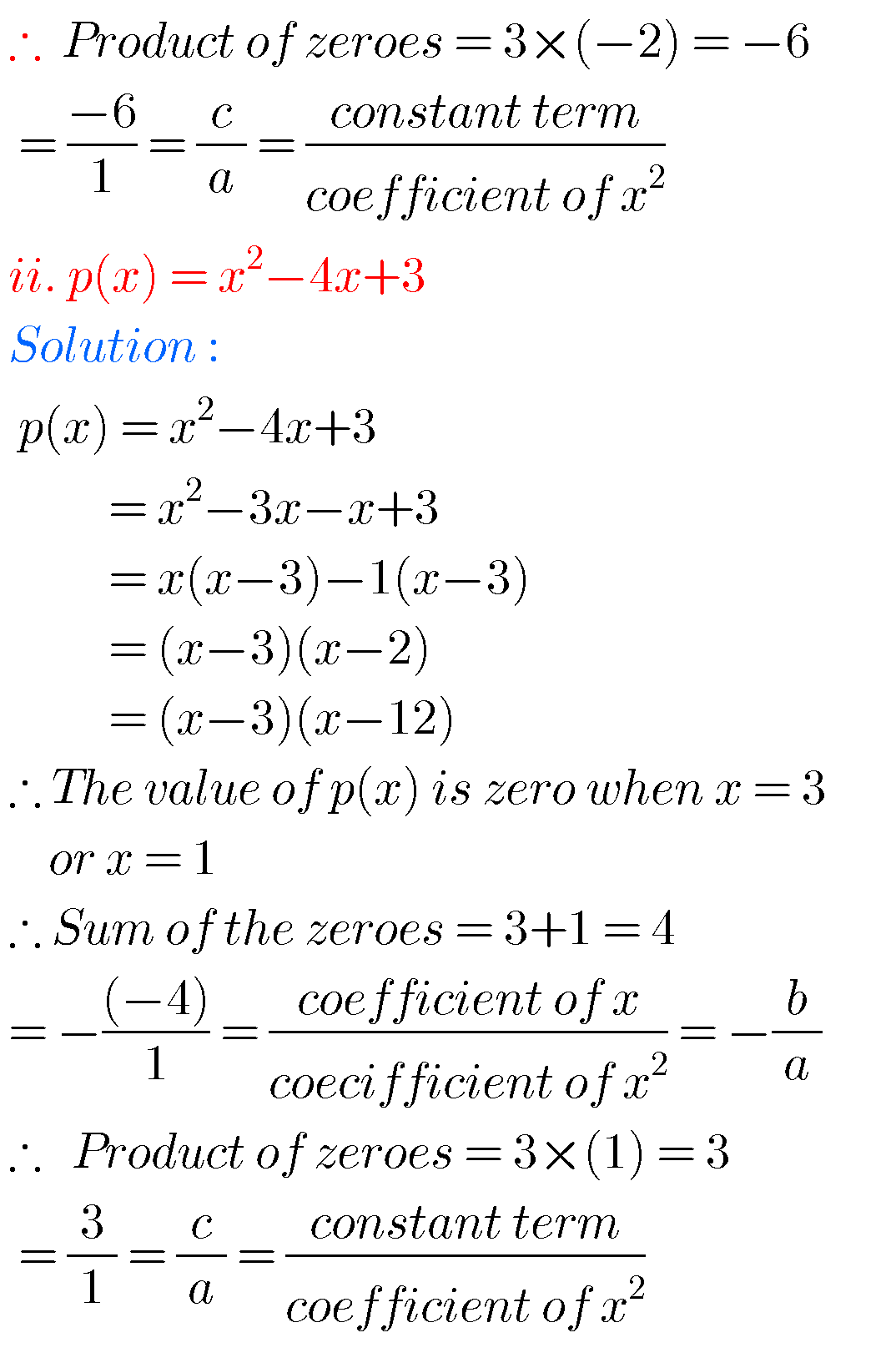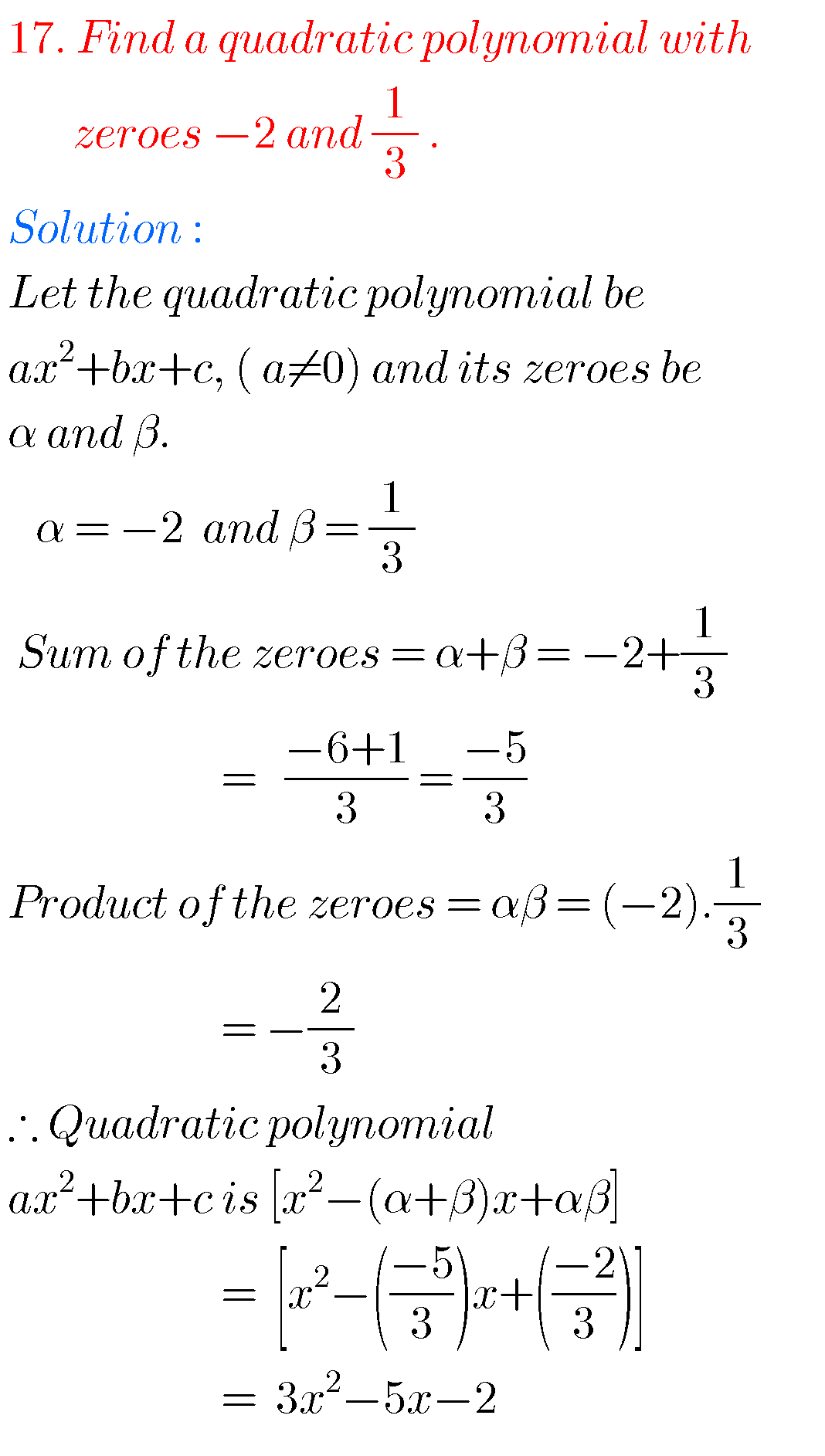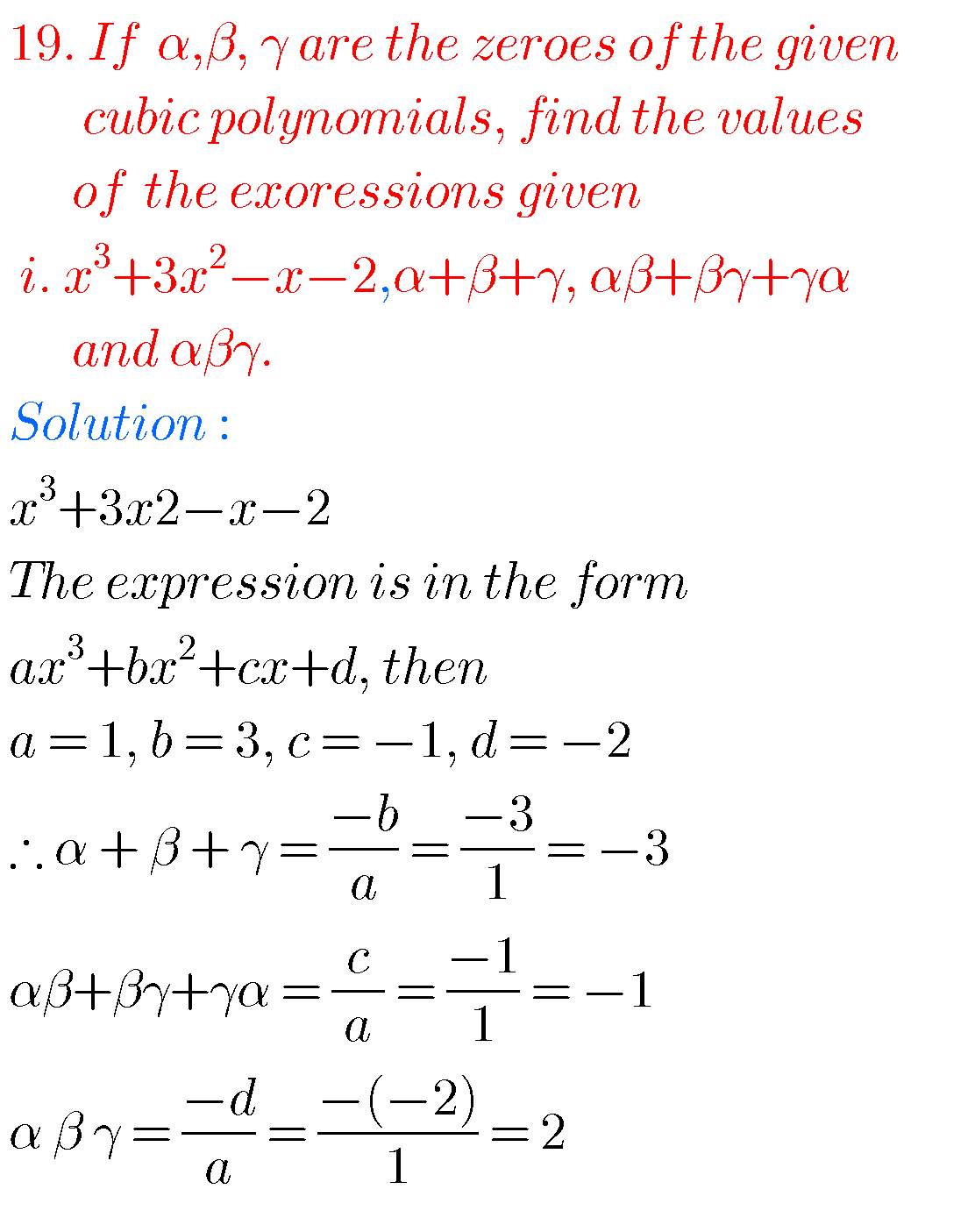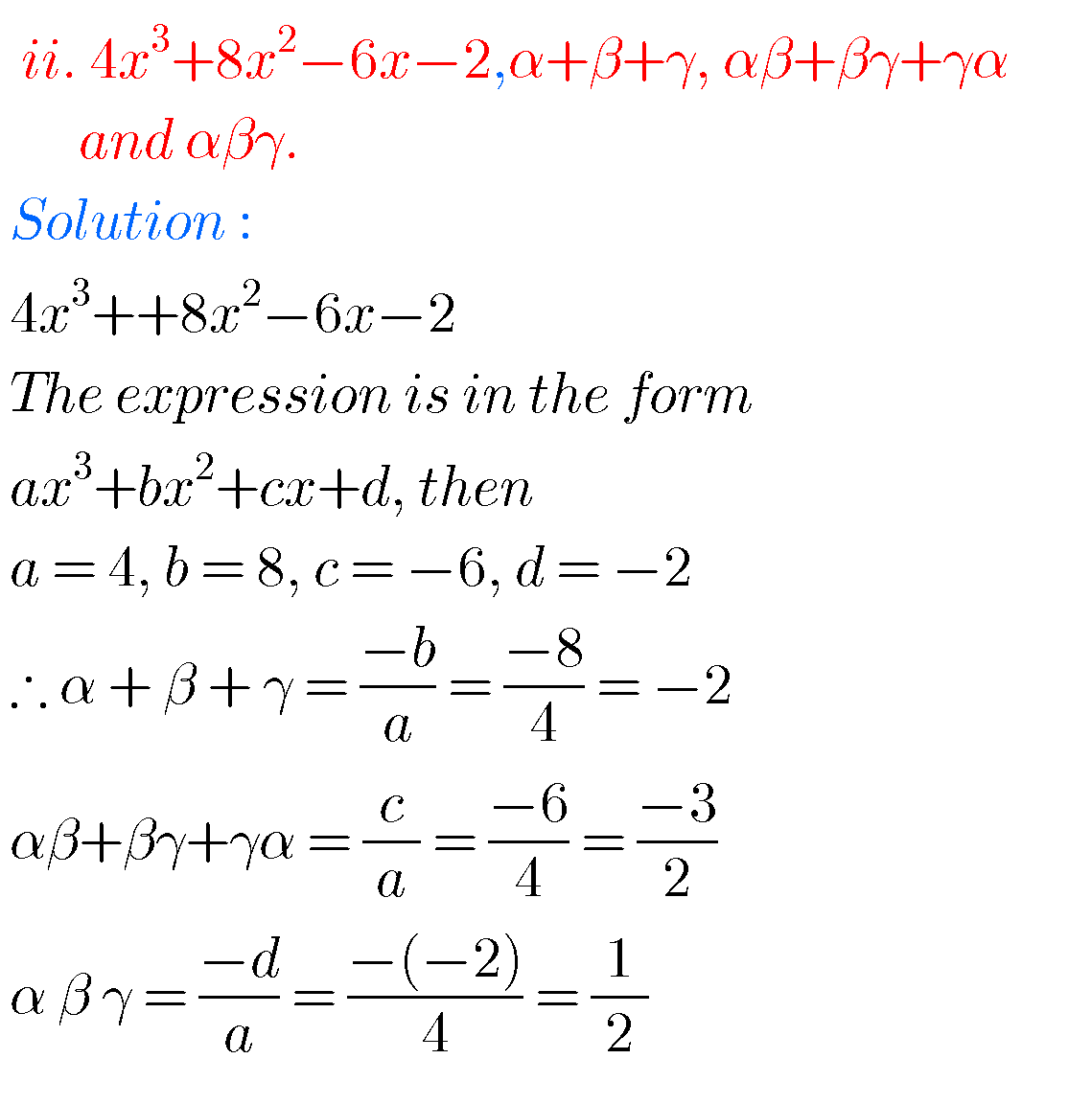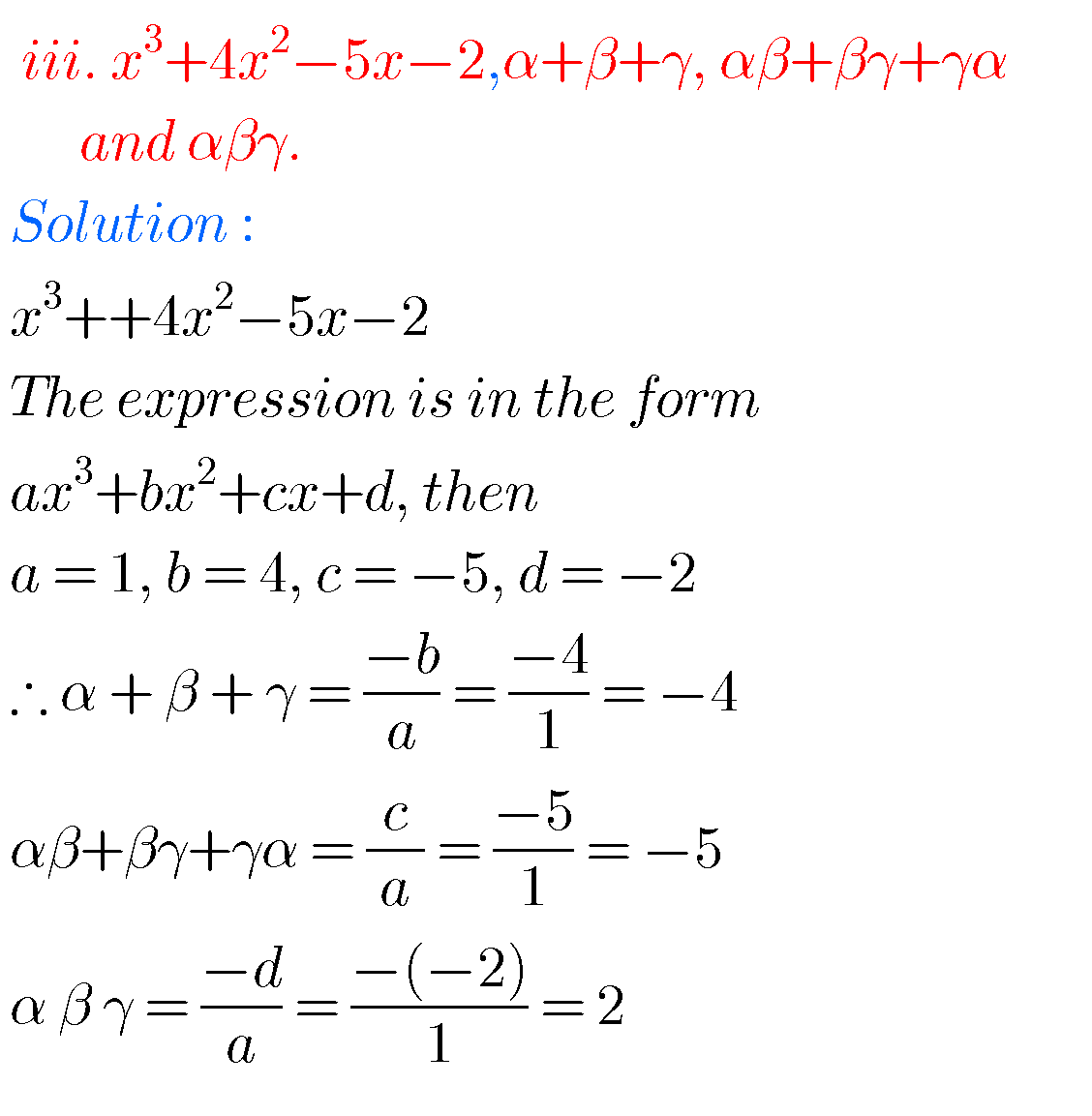Note :

Observe the solutions and try them in your own methods